# US6285509B1 - Zoom lens and display apparatus having the same - Google Patents

Zoom lens and display apparatus having the same Download PDF

## Info

Publication number
US6285509B1
US6285509B1 US09/407,077 US40707799A US6285509B1 US 6285509 B1 US6285509 B1 US 6285509B1 US 40707799 A US40707799 A US 40707799A US 6285509 B1 US6285509 B1 US 6285509B1
Authority
US
United States
Prior art keywords
lens unit
lens
wide
lt
angle end
Prior art date
Legal status (The legal status is an assumption and is not a legal conclusion. Google has not performed a legal analysis and makes no representation as to the accuracy of the status listed.)
Application number
US09/407,077
Inventor
Hiroki Nakayama
Akihiro Nishio
Saburo Sugawara
Current Assignee (The listed assignees may be inaccurate. Google has not performed a legal analysis and makes no representation or warranty as to the accuracy of the list.)
Canon Inc
Original Assignee
Canon Inc
Priority date (The priority date is an assumption and is not a legal conclusion. Google has not performed a legal analysis and makes no representation as to the accuracy of the date listed.)
Filing date
Publication date
Priority to JP9-367119 priority Critical
Priority to JP36711997A priority patent/JP4006073B2/en
Priority to JP10-296052 priority
Priority to JP29605298A priority patent/JP2000111797A/en
Priority to US21793998A priority
Priority to JP273299A priority patent/JP2000206409A/en
Priority to JP11-002732 priority
Priority to US09/407,077 priority patent/US6285509B1/en
Application filed by Canon Inc filed Critical Canon Inc
Assigned to CANON KABUSHIKI KAISHA reassignment CANON KABUSHIKI KAISHA ASSIGNMENT OF ASSIGNORS INTEREST (SEE DOCUMENT FOR DETAILS). Assignors: NAKAYAMA, HIROKI, NISHIO, AKIHIRO, SUGAWARA, SABURO
Application granted granted Critical
Publication of US6285509B1 publication Critical patent/US6285509B1/en
Anticipated expiration legal-status Critical
Application status is Expired - Lifetime legal-status Critical

## Images

•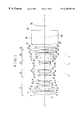•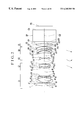•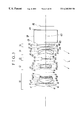•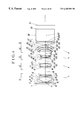•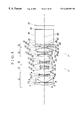•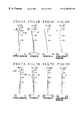•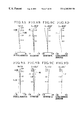•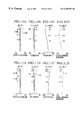•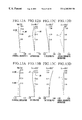•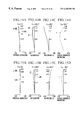•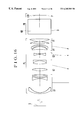•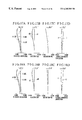•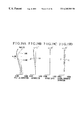•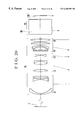•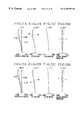•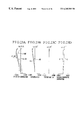•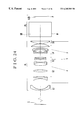•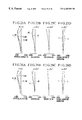•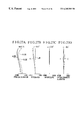•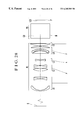•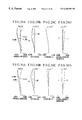•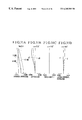•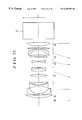•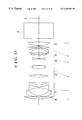•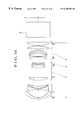•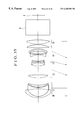•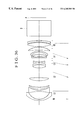•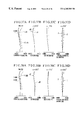•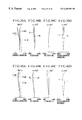•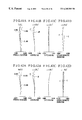•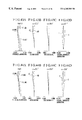•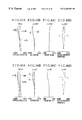••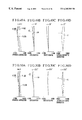•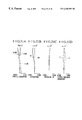•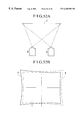## Classifications

• GPHYSICS
• G02OPTICS
• G02BOPTICAL ELEMENTS, SYSTEMS, OR APPARATUS
• G02B15/00Optical objectives with means for varying the magnification
• G02B15/14Optical objectives with means for varying the magnification by axial movement of one or more lenses or groups of lenses relative to the image plane for continuously varying the equivalent focal length of the objective
• G02B15/16Optical objectives with means for varying the magnification by axial movement of one or more lenses or groups of lenses relative to the image plane for continuously varying the equivalent focal length of the objective with interdependent non-linearly related movements between one lens or lens group, and another lens or lens group
• G02B15/177Optical objectives with means for varying the magnification by axial movement of one or more lenses or groups of lenses relative to the image plane for continuously varying the equivalent focal length of the objective with interdependent non-linearly related movements between one lens or lens group, and another lens or lens group having a negative front lens or group of lenses

## Abstract

A zoom lens for projecting an original image onto a screen in an enlarged scale includes, in order from the screen side, a first lens unit of negative refractive power, a second lens unit of positive refractive power, a third lens unit of negative refractive power, a fourth lens unit of positive refractive power and a fifth lens unit of positive refractive power, wherein, during zooming from a wide-angle end to a telephoto end, the second lens unit and the fourth lens unit move toward the screen side.

## Description

CROSS-REFERENCE TO RELATED APPLICATIONS

This application is a continuation-in-part of application Ser. No. 09/217,939 filed Dec. 22, 1998 abandoned.

BACKGROUND OF THE INVENTION

1. Field of the Invention

The present invention relates to zoom lenses suited to be used in display apparatuses, for example, projection apparatuses for displaying an original image onto a screen in an enlarged scale and, more particularly, to a zoom lens of simple design in a compact form suited to be used in a projection apparatus of the type in which three images of original colors on individual liquid crystal display panels or like displays are combined to form the original image in high definition and are then projected by only one projection lens.

2. Description of Related Art

The zoom lens whose front lens unit is negative in refractive power, that is, of the negative-lead type, has the advantages that the maximum field angle is relatively easy to increase and the image quality is kept stable in close-up photography. On the other hand, the zoom lens of the negative-lead type the such disadvantages that the zooming movement becomes longer and the zooming range is difficult to increase.

With these disadvantages in mind, zoom lenses of an increased range, while still permitting the bulk and size of the entire system to be reduced to a compact form, are disclosed in, for example, Japanese Patent Publication No. Sho 49-23912 and Japanese Laid-Open Patent Applications No. Sho 53-34539, No. Sho 57-163213, No. Sho 58-4113, No. Sho 63-241511 and No. Hei 2-201310.

In these publications, the zoom lens comprises four lens units of minus-plus-minus-plus refractive power arrangement in this order from the object side. Of the four lens units, predetermined ones are made to axially move in proper relation to effect zooming.

It is known to provide an apparatus for displaying an image on a screen in an enlarged scale by projection, in particular, an apparatus of a type in which a plurality of images of original colors on the respective individual liquid crystal display panels are combined to form an image in the gamut of colors, which is then projected by the projection lens. To adapt the negative-lead type zoom lenses mentioned above for application to such a projection lens, it becomes necessary to satisfy the following various conditions.

(i) The liquid crystal display panel has radiation characteristics and the assembly of dichroic mirrors suffers angle dependence in combining the plurality of images of original colors. In order to remove the influence of either of these factors, the optical system to be used should have the pupil as viewed from the display panel (the pupil as viewed from a shorter conjugate point side) put at a remote distance, or should be a so-called telecentric one.

(ii) The back focal distance should be long enough to secure the space the color combining elements occupy between the display panel assembly and the projection lens.

(iii) The image-bearing light beam is usually skewed upward as is projected onto the screen. So, the display panel assembly is arranged with its center shifted out of the optical axis. As a result, in a region near the front lens members, the effective area to use is not symmetrical with respect to the optical axis, but deviates upward. To allow this, the diameter of the front lens members necessarily becomes large. This must be obviated to reduce the bulk and size of the front lens members as far as possible.

Regardless of such requirements, in the prior art as previously mentioned, the pupil as viewed from a shorter conjugate point side lies at a finite distance and the back focal distance, too, cannot be said to be long enough.

BRIEF SUMMARY OF THE INVENTION

It is an object of the invention to eliminate the above-described problems and to provide a zoom lens suited to the projection lens in the display apparatus.

To attain the above object, in accordance with an aspect of the invention, there is provided a zoom lens for projecting an original image onto screen in an enlarged scale, which comprises, in order from the screen side, a first lens unit of negative refractive power, a second lens unit of positive refractive power, a third lens unit of negative refractive power, a fourth lens unit of positive refractive power and a fifth lens unit of positive refractive power, wherein, during zooming from a wide-angle end to a telephoto end, the second lens unit and the fourth lens unit move toward the screen side.

The above and further objects and features of the invention will become apparent from the following detailed description of preferred embodiments thereof taken in conjunction with the accompanying drawings.

BRIEF DESCRIPTION OF THE SEVERAL VIEWS OF THE DRAWING

FIG. 1 is a longitudinal section view of a first embodiment of the zoom lens according to the invention.

FIG. 2 is a longitudinal section view of a second embodiment of the zoom lens according to the invention.

FIG. 3 is a longitudinal section view of a third embodiment of the zoom lens according to the invention.

FIG. 4 is a longitudinal section view of a fourth embodiment of the zoom lens according to the invention.

FIG. 5 is a longitudinal section view of a fifth embodiment of the zoom lens according to the invention.

FIGS. 6A to 6D are graphic representations of the aberrations of a numerical example 1 of the zoom lens at the wide-angle end.

FIGS. 7A to 7D are graphic representations of the aberrations of the numerical example 1 of the zoom lens at the telephoto end.

FIGS. 8A to 8D are graphic representations of the aberrations of a numerical example 2 of the zoom lens at the wide-angle end.

FIGS. 9A to 9D are graphic representations of the aberrations of the numerical example 2 of the zoom lens at the telephoto end.

FIGS. 10A to 10D are graphic representations of the aberrations of a numerical example 3 of the zoom lens at the wide-angle end.

FIGS. 11A to 11D are graphic representations of the aberrations of the numerical example 3 of the zoom lens at the telephoto end.

FIGS. 12A to 12D are graphic representations of the aberrations of a numerical example 4 of the zoom lens at the wide-angle end.

FIGS. 13A to 13D are graphic representations of the aberrations of the numerical example 4 of the zoom lens at the telephoto end.

FIGS. 14A to 14D are graphic representations of the aberrations of a numerical example 5 of the zoom lens at the wide-angle end.

FIGS. 15A to 15D are graphic representations of the aberrations of the numerical example 5 of the zoom lens at the telephoto end.

FIG. 16 is a schematic diagram showing the main parts of a projection apparatus having a zoom lens according to a sixth embodiment of the invention.

FIGS. 17A to 17D are graphic representations of the aberrations of the zoom lens of a numerical example 6 in the wide-angle end.

FIGS. 18A to 18D are graphic representations of the aberrations of the zoom lens of the numerical example 6 in an intermediate focal length position.

FIGS. 19A to 19D are graphic representations of the aberrations of the zoom lens of the numerical example 6 in the telephoto end.

FIG. 20 is a schematic diagram showing the main parts of a projection apparatus having a zoom lens according to a seventh embodiment of the invention.

FIGS. 21A to 21D are graphic representations of the aberrations of the zoom lens of a numerical example 7 in the wide-angle end.

FIGS. 22A to 22D are graphic representations of the aberrations of the zoom lens of the numerical example 7 in an intermediate focal length position.

FIGS. 23A to 23D are graphic representations of the aberrations of the zoom lens of the numerical example 7 in the telephoto end.

FIG. 24 is a schematic diagram showing the main parts of a projection apparatus having a zoom lens according to an eighth embodiment of the invention.

FIGS. 25A to 25D are graphic representations of the aberrations of the zoom lens of a numerical example 8 at the wide-angle end.

FIGS. 26A to 26D are graphic representations of the aberrations of the zoom lens of the numerical example 8 in an intermediate focal length position.

FIGS. 27A to 27D are graphic representations of the aberrations of the zoom lens of the numerical example 8 at the telephoto end.

FIG. 28 is a schematic diagram showing the main parts of a projection apparatus having a zoom lens according to a ninth embodiment of the invention.

FIGS. 29A to 29D are graphic representations of the aberrations of the zoom lens of a numerical example 9 at the wide-angle end.

FIGS. 30A to 30D are graphic representations of the aberrations of the zoom lens of the numerical example 9 in an intermediate focal length position.

FIGS. 31A to 31D are graphic representations of the aberrations of the zoom lens of the numerical example 9 at the telephoto end.

FIG. 32 is a longitudinal section view of a tenth embodiment of the zoom lens according to the invention.

FIG. 33 is a longitudinal section view of an eleventh embodiment of the zoom lens according to the invention.

FIG. 34 is a longitudinal section view of a twelfth embodiment of the zoom lens according to the invention.

FIG. 35 is a longitudinal section view of a thirteenth embodiment of the zoom lens according to the invention.

FIG. 36 is a longitudinal section view of a fourteenth embodiment of the zoom lens according to the invention.

FIGS. 37A to 37D are graphic representations of the various aberrations of the zoom lens of a numerical example 10 at the wide-angle end.

FIGS. 38A to 38D are graphic representations of the various aberrations of the zoom lens of the numerical example 10 in an intermediate focal length position.

FIGS. 39A to 39D are graphic representations of the aberrations of the zoom lens of the numerical example 10 at the telephoto end.

FIGS. 40A to 40D are graphic representations of the various aberrations of the zoom lens of a numerical example 11 at the wide-angle end.

FIGS. 41A to 41D are graphic representations of the various aberrations of the zoom lens of the numerical example 11 in an intermediate focal length position.

FIGS. 42A to 42D are graphic representations of the aberrations of the zoom lens of the numerical example 11 at the telephoto end.

FIGS. 43A to 43D are graphic representations of the various aberrations of the zoom lens of a numerical example 12 at the wide-angle end.

FIGS. 44A to 44D are graphic representations of the various aberrations of the zoom lens of the numerical example 12 in an intermediate focal length position.

FIGS. 45A to 45D are graphic representations of the aberrations of the zoom lens of the numerical example 12 at the telephoto end.

FIGS. 46A to 46D are graphic representations of the various aberrations of the zoom lens of a numerical example 13 at the wide-angle end.

FIGS. 47A to 47D are graphic representations of the various aberrations of the zoom lens of the numerical example 13 in an intermediate focal length position.

FIGS. 48A to 48D are graphic representations of the aberrations of the zoom lens of the numerical example 13 at the telephoto end.

FIGS. 49A to 49D are graphic representations of the various aberrations of the zoom lens of a numerical example 14 in the wide-angle end.

FIGS. 50A to 50D are graphic representations of the various aberrations of the zoom lens of the numerical example 14 in an intermediate focal length position.

FIGS. 51A to 51D are graphic representations of the aberrations of the zoom lens of the numerical example 14 at the telephoto end.

FIGS. 52A and 52B are plan views for explaining the picture element offset at the time of stack projection.

DETAILED DESCRIPTION OF THE INVENTION

Hereinafter, preferred embodiments of the invention will be described in detail with reference to the drawings.

FIG. 1 is a longitudinal section view of a first embodiment of the zoom lens according to the invention, which is used to project image information of a display element PI (original image) in an enlarged scale onto a screen (not shown) that is assumed to be present on the left side at a far distance. In the first embodiment, the zoom lens comprises, in order from the screen side (the left side as viewed in FIG. 1), a first lens unit L1 of negative refractive power, a second lens unit L2 of positive refractive power, a third lens unit L3 of negative refractive power, a fourth lens unit L4 of positive refractive power and a fifth lens unit L5 of positive refractive power, followed by a color-combining element DP such as a dichroic prism. Further, in the first embodiment, to simplify the structural arrangement of the zoom lens, the first lens unit L1 and the fifth lens unit L5 are made stationary during zooming. Of the remaining lens units, the second lens unit L2 and the fourth lens unit L4 axially move toward the screen side to effect zooming from the wide-angle end to the telephoto end, while the third lens unit L3 simultaneously moves in a locus convex toward the screen side to compensate for the shift of an image plane with the movements of the second lens unit L2 and the fourth lens unit L4. Accordingly, during zooming from the wide-angle end to the telephoto end, the separation between the first lens unit L1 and the second lens unit L2 decreases, the separation between the second lens unit L2 and the third lens unit L3 increases, the separation between the third lens unit L3 and the fourth lens unit L4 decreases, and the separation between the fourth lens unit L4 and the fifth lens unit L5 increases. Although, in FIG. 1, only one display element PI is illustrated, in actual practice that there are a plurality of display elements corresponding to the original colors such as red, green and blue. The light beams from the plurality of display elements are combined in passing through the color combining element DP to form an image, which is then projected by the zoom lens onto the screen.

In the present embodiment, the fifth lens unit L5, which is closest to the display element PI, is given a relatively strong positive refractive power to realize formation of a telecentric system toward the display element PI. Still another feature of the first embodiment is that the fifth lens unit L5 is made up from one positive lens having a lens surface facing the screen side, which is stronger in convex curvature than a lens surface thereof facing the display element PI, thereby achieving simultaneous fulfillment of the correction of off-axial curvature of field and of the simplification of the structural arrangement. Further, it is preferred that the fifth lens unit L5 has at least one bi-convex lens, as in the present embodiment.

By making the zooming provision in the second lens unit L2, the third lens unit L3 and the fourth lens unit L4, the total movement of each of the second lens unit L2 and the fourth lens unit L4 is invaluably reduced, while still maintaining a great increase of the zooming range to be achieved, thus assuring that the total length of the entire lens system is to be shortened and, at the same time, that, as the position of the entrance pupil determines the diameter of the front lens members which admit of the off-axial oblique light beam, its distance from the front vertex, too, is shortened.

The first lens unit L1 is made negative in refractive power to secure a long back focal distance for the space the color combining element DP occupies. In particular, to increase the back focal distance, the first lens unit L1 is better made to include a negative meniscus lens convex toward the screen side. Further, all the lens units have their refractive powers in an appropriate arrangement and the first lens unit L1 is made stationary during zooming, thereby making it possible to realize a lens system that varies the position of the off-axial oblique light beam to a lesser extent and is simple to design and still whose total length is kept constant during zooming. Also, to reduce distortion at the wide-angle end, a convex lens is put at the position closest to the screen side in the first lens unit L1 to admit of the most off-axial light beam in correcting distortion.

Focusing onto finite distances is preferably carried out by the first lens unit L1. However, the third lens unit L3 or the fifth lens unit L5 may be used instead. Focusing may be otherwise carried out by moving two or more of the lens units in differential relation. Focusing can also be carried out by bodily moving the zoom lens or adjusting the position of the screen.

In the first embodiment shown in FIG. 1, during zooming from the wide-angle end to the telephoto end, the first lens unit L1 and the fifth lens unit L5 remain stationary, the second lens unit L2 and the fourth lens unit L4 axially move toward the screen side, while the third lens unit L3 axially moves in a locus convex toward the screen side with the inflection occurring at a nearer point to the telephoto end. The first embodiment provides an arrangement for the largest relative aperture among all the embodiments.

FIG. 2 shows a second embodiment of the zoom lens, where, similarly to the first embodiment, during zooming from the wide-angle end to the telephoto end, the first lens unit L1 and the fifth lens unit L5 remain stationary and the second lens unit L2 and the fourth lens unit L4 axially move toward the screen side, while the third lens unit L3 axially moves in a locus convex toward the screen side with the inflection occurring at a nearer point to the telephoto end. This zoom lens has a higher zoom ratio.

FIG. 3 shows a third embodiment of the zoom lens, which is different in structure from the second embodiment. In the third embodiment, during zooming from the wide-angle end to the telephoto end, the first lens unit L1 and the fifth lens unit L5 remain stationary and the second lens unit L2 and the fourth lens unit L4 axially move toward the screen side, while the third lens unit L3 axially moves in a locus convex toward the screen side with the inflection occurring at a nearer point to the telephoto end.

FIG. 4 shows a fourth embodiment of the zoom lens. In the fourth embodiment, during zooming from the wide-angle end to the telephoto end, the first lens unit L1 axially moves in a locus convex toward the screen side, taking a nearer position to the display element PI side in the telephoto end than at the wide-angle end. The fifth lens unit L5 remains stationary during zooming. Also, during zooming from the wide-angle end to the telephoto end, the second lens unit L2 and the fourth lens unit L4 axially move toward the screen side, while the third lens unit L3 axially moves in a locus convex toward the screen side with the inflection occurring at a nearer point to the telephoto end.

FIG. 5 shows a fifth embodiment of the zoom lens, where, during zooming from the wide-angle end to the telephoto end, the first lens unit L1 and the fifth lens unit L5 remain stationary and the second lens unit L2 and the fourth lens unit L4 axially move toward the screen side. The third lens unit L3, too, remains stationary during zooming.

In the above-described embodiments, for more desired results, it is preferred that the focal lengths f1 and f2 of the first lens unit L1 and the second lens unit L2, respectively, satisfy the following conditions: $1.1 <  f1  / f2 < 2.3 ( 1 ) 0.6 < f2 / fw · ft < 1.2 ( 2 )$

where fw and ft are the focal lengths at the wide-angle end and the telephoto end of the entire lens system, respectively.

The inequalities of condition (1) give a proper range for the correlation of the second lens unit L2 as the main variator with the first lens unit L1. When the lower limit of the condition (1) is exceeded, the diameter of the front members, which is determined depending on the focal length f1, becomes large and the distortion increases at the wide-angle end. So, these are objectionable. When the upper limit is exceeded, the total movement of the second lens unit L2 has to increase. Otherwise, the desired zoom ratio could not be obtained. Therefore, the size of the entire lens system is caused to increase objectionably.

The inequalities of condition (2) give a proper range for the refractive power of the main variator. When the lower limit is exceeded, over-correction of filed curvature results. When the upper limit is exceeded, the total movement of the second lens unit L2 increases to obtain the desired zoom ratio and a large increase of the size of the entire lens system results. So, these are objectionable.

In particular, to correct distortion well, it is preferred to satisfy the following condition:

1<|f1|/fw<2  (3)

When the upper limit of the condition (3) is exceeded, the distortion in the wide-angle end can no longer be corrected well. When the lower limit is exceeded, the distortion in the telephoto end can no longer be corrected well.

It is to be noted that the term “nearly telecentric” or “just telecentric” system used in the present specification means that, since, as described before, the liquid crystal display has a radiation characteristics and the combination of colors depends on the angles of inclination of the dichroic mirrors, the system in question has its exit pupil made to lie at a remote distance (ideally at infinity), thus removing the influence of either of them. In actual practice, for that angle dependency to be taken into account, it is preferred that, of the absolute values of the distance from the display panel (contracted side) to the exit pupil over the entire zooming range, the minimum distance |tk| lies in the following range:

|tk|/fw>4.0  (4)

For more desired results, it is preferred to alter the condition (4) as follows:

|tk|/fw>9.0  (4′)

For the main variator or second lens unit L2, letting the ratio of the magnification β2t at the telephoto end of the second lens unit L2 to the magnification β2w at the wide-angle end of the second lens unit L2 be denoted by Z2, the ratio of the focal length ft at the telephoto end of the entire lens system to the focal length fw at the wide-angle end of the entire lens system be denoted by Z, and the absolute values of total zooming movement of the second lens unit L2 and the fourth lens unit L4 be denoted by M2 and M4, respectively, it is preferred to satisfy the following conditions: $0.8 < Z2 / Z < 1.1 ( 5 ) 0.9 < M2 / M4 < 1.6 ( 6 ) 0.4 < M2 / ( ft - fw ) < 1.0 ( 7 )$

The inequalities of condition (5) give a proper range for the ratio of the variable magnifications of the second lens unit L2 and the fourth lens unit L4, which serve as variators. Because the third lens unit L3 contributes to a decrease of the variable magnification, it is preferred to retain the above range. The inequalities of conditions (6) and (7) have an aim to make a good compromise between the total length of the entire lens system and the zooming movement of each of the variators. In particular, of the second lens unit L2 and the fourth lens unit L4, the fourth lens unit L4 is rather apt to have a weak refractive power. To assure the contribution of the fourth lens unit L4 to an appropriate variation of the focal length, it is preferred to retain the above range. In particular, it is more preferred that the total zooming movement of the second lens unit L2 exceeds the total zooming movement of the fourth lens unit L4.

On consideration of the fact that, as described above, the fourth lens unit L4 is more apt to become weak in refractive power than the second lens unit L2 is, it is preferred to satisfy particularly the following condition:

0.4<f2/f4<0.8  (8)

where f4 is the focal length of the fourth lens unit L4.

The inequalities of conditions (8) and (6) are necessary ones for making an appropriate power arrangement and variation of the main variator to determine a proper value of the Petzval sum.

To put the exit pupil of the entire lens system at a proper distance and make acceptable distortion, letting the distance from the fifth lens unit L5 to the display element PI, as reduced to air by removing the dichroic prism DP, be denoted by bf, and the focal length of the fifth lens unit L5 be denoted by f5, it is preferred to satisfy the following conditions: $0.3 < bf / f5 < 0.5 ( 9 ) 1.2 <  f1  / bf < 2.2 ( 10 )$

The inequalities of condition (9) are necessary ones for making the entire lens system to a proper telecentric form. When the upper limit is exceeded, the entire lens system increases in size greatly. When the lower limit is exceeded, distortion is produced. The inequalities of condition (10) are also for elongating the exit pupil to insure formation of the telecentric system in such a manner that the distortion produced is acceptable.

In particular, with the telecentric system optimumly formed, in order to optimize the distance from the zoom lens to the display panel, it is preferred to satisfy the following condition:

2<f5/fw<3.5  (11)

When the lower limit of the condition (11) is exceeded, the optimum telecentricity cannot be satisfied. When the upper limit is exceeded, the size increases objectionably.

To further improve the compact form, the refractive powers of all the lens units except the main variator must be appropriately arranged to make proper the movements of the zooming lens units. For this purpose, it is preferred to satisfy the following conditions: $1.0 <  f1  / fw · ft < 1.6 ( 12 ) 0.6 <  f3  / fw · ft < 1.2 ( 13 ) 1.1 < f4 / fw · ft < 1.8 ( 14 ) 1.5 < f5 / fw · ft < 3.0 ( 15 )$

where f3 is the focal length of the third lens unit L3.

Particularly for lateral chromatic aberration, not only to reduce it over the entire zooming range, but also to maintain it stable, it is preferred that the third lens unit L3 includes a lens element whose Abbe number ν3 lies within the following range:

ν3>55  (16)

In particular, it is more preferred to narrow the range as follows:

ν3>60  (16′)

Also, in order to decrease chromatic aberrations over the entire zooming range and reduce the range of variation of the chromatic aberrations due to zooming, the first lens unit L1 has to be constructed with inclusion of negative lens elements whose mean Abbe number ν1n lies within the following range:

ν1n>60  (17)

Next, five numerical examples 1 to 5 corresponding to the first to fifth embodiments, respectively, are shown. In the numerical data for the examples 1 to 5, ri is the radius of curvature of the i-th surface, when counted from the screen side, di is the i-th lens thickness or air separation, when counted from the screen side, and ni and νi are respectively the refractive index and Abbe number of the material of the i-th optical element, when counted from the screen side.

Numerical Example 1:

 f = 100.00000˜129.3 Fno = 1:1.8˜2.1 2ω= 55°˜43.6° r1 = 257.877 d1 = 21.15 n1 = 1.51633 v1 = 64.1 r2 = −916.765 d2 = 0.60 r3 = 173.203 d3 = 6.63 n2 = 1.51633 v2 = 64.1 r4 = 76.580 d4 = 32.14 r5 = −146.753 d5 = 6.03 n3 = 1.51633 v3 = 64.1 r6 = 100.187 d6 = 22.59 r7 = 136.079 d7 = 9.05 n4 = 1.80518 v4 = 25.4 r8 = 226.085 d8 = Variable r9 = 458.458 d9 = 8.52 n5 = 1.77250 v5 = 49.6 r10 = −458.585 d10 = 11.97 r11 = 230.660 d11 = 20.02 n6 = 1.69680 v6 = 55.5 r12 = −92.414 d 12= 4.22 n7 = 1.80518 v7 = 25.4 r13 = −204.747 D13 = Variable (Stop) r14 = −117.639 d14 = 3.92 n8 = 1.51633 v8 = 64.1 r15 = 117.639 d15 = 9.65 n9 = 1.80518 v9 = 25.4 r16 = 145.223 d16 = Variable r17 = −443.581 d17 = 31.26 n10 = 1.69680 v10 = 55.5 r18 = −64.074 d18 = 6.03 n11 = 1.80518 v11 = 25.4 r19 = −131.339 d19 = 0.60 r20 = 2090.520 d20 = 16.52 n12 = 1.60311 v12 = 60.6 r21 = −220.342 d21 = Variable r22 = 186.134 d22 = 18.37 n13 = 1.60311 v13 = 60.6 r23 = −4345.838 d23 = 20.25 r24 = ∞ d24 = 96.48 n14 = 1.51633 v14 = 64.2 r25 = ∞ Variable Focal Length Separation 100.00 113.61 129.30 d8 34.64 20.45 9.16 d13 42.21 50.54 61.65 d16 27.65 22.84 14.98 d21 3.02 13.68 21.73

Numerical Example 2:

 f = 100.00000˜158.97 Fno = 1:2.3˜3.0 2ω= 50.5°32.3° r1 = 249.961 d1 = 12.42 n1 = 1.60311 v1 = 60.6 r2 = −411.372 d2 = 0.55 r3 = 205.115 d3 = 4.42 n2 = 1.51633 v2 = 64.1 r4 = 58.412 d4 = 26.29 r5 = −76.525 d5 = 4.14 n3 = 1.51633 v3 = 64.1 r6 = 273.991 d6 = 1.38 r7 = 144.155 d7 = 7.18 n4 = 1.80518 v4 = 25.4 r8 = 477.185 d8 = Variable r9 = 238.906 d9 = 7.45 n5 = 1.83400 v5 = 37.2 r10 = −238.906 d10 = 0.41 r11 = 236.764 d11 = 16.01 n6 = 1.72000 v6 = 50.2 r12 = −56.537 d12 = 3.31 n7 = 1.76182 v7 = 26.5 r13 = −373.800 D13 = Variable (Stop) r14 = −112.532 d14 = 3.31 n8 = 1.48749 v8 = 70.2 r15 = 112.532 d15 = Variable r16 = −189.788 d16 = 17.94 n9 = 1.69680 v9 = 55.5 r17 = −59.517 d17 = 2.76 r18 = −58.018 d18 = 4.97 n10 = 1.80518 v10 = 25.4 r19 = −105.595 d19 = 1.38 r20 = −1913.013 d20 = 11.04 n11 = 1.63854 v11 = 55.4 r21 = −201.683 d21 = Variable r22 = 205.826 d22 = 15.46 n12 = 1.63854 v12 = 55.4 r23 = −385.215 d23 = 9.66 r24 = ∞ d24 = 82.82 n13 = 1.51633 v13 = 64.2 r25 = ∞ Variable Focal Length Separation 100.00 130.63 158.97 d8 39.86 15.98 3.17 d13 27.30 41.55 53.99 d15 39.54 28.81 13.83 d21 2.76 23.12 38.48

Numerical Example 3:

 f = 100.00000˜158.06 Fno = 1:2.31˜3.0 2ω= 50.3°˜32.3° r1 = 196.239 d1 = 15.07 n1 = 1.60311 v1 = 60.6 r2 = −491.879 d2 = 0.55 r3 = 179.892 d3 = 4.41 n2 = 1.51633 v2 = 64.1 r4 = 55.314 d4 = 28.35 r5 = −74.931 d5 = 4.13 n3 = 1.51633 v3 = 64.1 r6 = 291.815 d6 = 0.10 r7 = 131.408 d7 = 7.17 n4 = 1.80518 v4 = 25.4 r8 = 352.793 d8 = Variable r9 = 231.211 d9 = 7.99 n5 = 1.83400 v5 = 37.2 r10 = −21.515 d10 = 0.48 r11 = 413.645 d11 = 15.16 n6 = 1.72000 v6 = 50.2 r12 = −55.333 d12 = 3.31 n7 = 1.76182 v7 = 26.5 r13 = −323.885 d13 = Variable (Stop) r14 = −98.340 d14 = 3.31 n8 = 1.48749 v8 = 70.2 r15 = 140.889 d15 = Variable r16 = −153.499 d16 = 19.30 n9 = 1.69680 v9 = 55.5 r17 = −54.452 d17 = 4.96 n10 = 1.80518 v10 = 25.4 r18 = −94.141 d18 = 0.83 r19 = 3043.936 d19 = 12.40 n11 = 1.63854 v11 = 55.4 r20 = −199.501 d20 = Variable r21 = 163.968 d21 = 17.37 n12 = 1.63854 v12 = 55.4 r22 = −21522.170 d22 = 25.52 r23 = ∞ d23 = 82.70 n13 = 1.51633 v13 = 64.2 r24 = ∞ Variable Focal Length Separation 100.00 124.72 158.06 d8 38.59 20.45 3.01 d13 27.71 41.43 60.14 d15 36.45 29.19 13.35 d20 2.76 16.57 28.99

Numerical Example 4:

 f = 100.00000˜154.3 Fno = 1:2.5˜3.0 2ω= 55.8°˜36.5° r1 = −1988.193 d1 = 9.69 n1 = 1.77250 v1 = 49.6 r2 = −296.881 d2 = 0.29 r3 = 186.766 d3 = 4.84 n2 = 1.48749 v2 = 70.2 r4 = 71.563 d4 = 29.18 r5 = −86.237 d5 = 4.54 n3 = 1.48749 v3 = 70.2 r6 = 431.800 d6 = 3.03 r7 = 370.629 d7 = 7.57 n4 = 1.84666 v4 = 23.8 r8 = −1187.571 d8 = Variable (Stop) r9 = 129.582 d9 = 9.69 n5 = 1.60311 v5 = 60.6 r10 = −263.492 d10 = 0.45 r11 = 152.292 d11 = 13.02 n6 = 1.69680 v6 = 55.5 r12 = −90.645 d12 = 3.63 n7 = 1.75520 v7 = 27.5 r13 = −887.546 D13 = Variable r14 = −134.654 d14 = 3.63 n8 = 1.63854 v8 = 55.4 r15 = 87.770 d15 = 2.97 r16 = 166.658 d16 = 6.66 n9 = 1.69680 v9 = 55.5 r17 = −963.346 d17 = 3.63 n10 = 1.80518 v10 = 25.4 r18 = 273.134 d18 = Variable r19 = −240.499 d19 = 13.63 n11 = 1.69680 v11 = 55.5 r20 = −96.487 d20 = 6.96 r21 = −62.030 d21 = 7.57 n12 = 1.74077 v12 = 27.8 r22 = −85.500 d22 = 1.57 r23 = −236.107 d23 = 12.11 n13 = 1.69680 v13 = 55.5 r24 = −106.664 d24 = Variable r25 = 174.522 d25 = 16.65 n14 = 1.69680 v14 = 55.5 r26 = −946.850 d26 = 4.54 r27 = ∞ d27 = 90.84 n15 = 1.51633 v15 = 64.2 r28 = ∞ Variable Focal Length Separation 100.00 118.18 154.34 d8 61.13 36.56 3.14 d13 29.66 33.09 44.81 d18 21.88 18.45 6.73 d24 3.38 19.22 31.16

Numerical Example 5:

 f = 100.00000˜150.15 Fno = 1:2.4˜3.0 2ω= 53.1°˜35.5° r1 = 172.840 d1 = 17.04 n1 = 1.74320 v1 = 49.3 r2 = −3756.301 d2 = 0.28 r3 = 238.065 d3 = 4.70 n2 = 1.48749 v2 = 70.2 r4 = 62.862 d4 = 37.62 r5 = −100.083 d5 = 4.41 n3 = 1.57099 v3 = 50.8 r6 = 172.304 d6 = 1.25 r7 = 125.526 d7 = 5.88 n4 = 1.84666 v4 = 23.8 r8 = 234.088 d8 = Variable r9 = 379.046 d9 = 7.35 n5 = 1.80400 v5 = 46.6 r10 = −169.885 d10 = 0.44 r11 = 135.550 d11 = 9.70 n6 = 1.74320 v6 = 49.3 r12 = −91.631 d12 = 3.53 n7 = 1.75520 v7 = 27.5 r13 = 403.913 D13 = Variable (Stop) r14 = −142.892 d14 = 3.53 n8 = 1.48749 v8 = 70.2 r15 = 107.820 d15 = 2.49 r16 = 119.454 d16 = 5.29 n9 = 1.84666 v9 = 23.8 r17 = 143.772 d17 = Variable r18 = 546.045 d18 = 15.20 n10 = 1.83481 v10 = 42.7 r19 = −103.896 d19 = 5.31 r20 = −88.135 d20 = 5.29 n11 = 1.76182 v11 = 26.5 r21 = 192.346 d21 = 6.57 r22 = 251.818 d22 = 24.10 n12 = 1.74320 v12 = 49.3 r23 = −137.445 d23 = Variable r24 = 472.968 d24 = 9.40 n13 = 1.74320 v13 = 49.3 r25 = −1071.045 d25 = 0.44 r26 = 191.838 d26 = 9.40 n14 = 1.74320 v14 = 49.3 r27 = 472.256 d27 = 10.29 r28 = ∞ d28 = 88.16 n15 = 1.51633 v15 = 64.2 r29 = ∞ Variable Focal Length Separation 100.00 136.30 150.15 d8 33.97 8.76 2.81 d13 17.54 42.76 48.71 d17 32.31 17.92 8.33 d23 2.93 17.32 26.91

The values of the design parameters and the factors of the conditions (1) to (15) for the numerical examples 1 to 5 are given in the tables below.

 Numerical Example 1 2 3 4 5 Parameter β2w −0.9887 −0.8267 −0.9129 −0.4975 −1.0119 β2t −1.2624 −1.2431 −1.3801 −0.7438 −1.5083 β4w −0.3192 −0.1594 −0.2908 0.099 −0.1118 β4t −0.4336 −0.3426 −0.4506 −0.0467 −0.2451 Z (= ft/fw) 1.293215 1.589747 1.580577 1.543439 1.501484 Z2 (= β2t/2w) 1.276828 1.503689 1.511776 1.495075 1.490562 Z4 (=β4t/β4w) 1.358396 2.14931 1.549519 −0.47172 2.192308 Condition No. & Factor (12) $ f1  / fw · ft$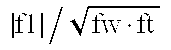1.31352 1.1461 1.2043 1.41822 1.25413 (2) $f2 / fw · ft$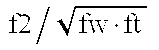1.021893 0.718257 0.763224 0.701503 0.78199 (13) $ f3  / fw · ft$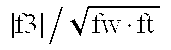1.16191 0.91104 0.9407 0.72778 1.20923 (14) $f4 / fw · ft$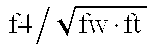1.439296 1.546381 1.30547 1.534361 1.469586 (15) $f5 / fw · ft$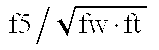2.606882 1.683398 2.027713 1.712783 1.77807 (8) f2/f4 0.709995 0.464476 0.584635 0.457196 0.532116 (3) |f1|/fw 1.49373 1.44506 1.51406 1.76192 1.53675 (1) |f1|/f2 1.28538 1.59567 1.57791 2.02168 1.60377 (5) Z2/Z 0.987329 0.945867 0.956471 0.968665 0.992726 (6) M2/M4 1.361473 1.027484 1.356137 1 1.2995 (9) bf/f5 0.380981 0.48291 0.418234 0.409213 0.416409 (10) |f1|/bf 1.32255 1.40984 1.42007 2.02344 1.69384 (7) M2/(ft − fw) 0.869 0.62229 0.61283 0.51118 0.62143 (11) f5/fw 2.964536 2.122515 2.549261 2.127881 2.178759 (4) |tk|/fw 14.6866 9.25914 8.50135 13.7775 9.60034

FIGS. 6A to 6D through FIGS. 15A to 15D graphically show the various aberrations of the numerical examples 1 to 5 at the wide-angle end and the telephoto end, respectively.

It will be appreciated from the foregoing that the zoom lens of the invention is simple in design, fast in speed, compact in the bulk and size, high in the zoom ratio, small in distortion and smaller in the variation of lateral chromatic aberration. Therefore, the image of high definition can be projected with high accuracy and reliability.

Next, zoom lenses according to sixth to ninth embodiments of the invention will be described.

FIGS. 16, 20, 24 and 28 are schematic block diagrams of the main parts of projection apparatuses (liquid crystal video projectors) using numerical examples 6 to 9 of zoom lenses whose data will be given later in correspondence to the sixth to ninth embodiments of the invention, respectively. The aberrations of the zoom lenses of numerical examples 6 to 9 at the wide-angle end, an intermediate focal length and the telephoto end are shown in FIGS. 17A to 17D through FIGS. 19A to 19D, FIGS. 21A to 21D through FIGS. 23A to 23D, FIGS. 25A to 25D through FIGS. 27A to 27D and FIGS. 29A to 29D through FIGS. 31A to 31D.

In all the lens block diagrams, a zoom lens PL comprises a first lens unit L1 of negative refractive power, a second lens unit L2 of positive refractive power, a third lens unit L3 of negative refractive power, a fourth lens unit L4 of positive refractive power and a fifth lens unit L5 of positive refractive power.

A screen S (projection plane) and a liquid crystal display LCD (an original image to be projected) are positioned in conjugate relation. Usually, the screen S takes its place at a long conjugate point (first conjugate point) and the display LCD at a short conjugate point (second conjugate point). A glass block GB includes a color combining prism, a polarizing filter, a color filter and others.

The zoom lens PL is mounted in a projector body PB through a coupling member (not shown). The glass block GB and the display element LCD are contained in the projector body PB.

In the sixth to ninth embodiments, during zooming from the wide-angle end to the telephoto end, as indicated by the arrows, the second and fourth lens units L2 and L4 axially move toward the first conjugate point (toward the screen S), while simultaneously moving the third lens unit L3 either in a locus convex toward the first conjugate point, or monotonously toward the first conjugate point. The first lens unit L1 axially moves to effect focusing. Incidentally, focusing may otherwise be performed by moving the third or fifth lens unit, or the whole of the zoom lens.

In the projection apparatus of each of the sixth to ninth embodiments, the zoom lens PL is used to project the original image formed on the display LCD onto the surface of the screen S.

The features of the zoom lenses of the sixth to ninth embodiments are described below.

The zoom lens PL comprises, in order from the screen side (large conjugate side), the first lens unit of negative refractive power containing at least one aspheric surface, the second lens unit of positive refractive power, the third lens unit of negative refractive power, the fourth lens unit of positive refractive power and the fifth lens unit of positive refractive power, wherein, during zooming from the wide-angle end to the telephoto end, the separation between the first and second lens units decreases, the separation between the second and third lens units increases, the separation between the third and fourth lens units decreases and the separation between the fourth and fifth lens units increases. Preferably, for the purpose of simplifying the structure of construction, the first and fifth lens units are made fixed. Of the remaining lens units, the second and fourth lens units are made to move toward the screen side to effect zooming. The third lens unit moves to compensate for the shift of an image plane with the zooming of the second and fourth lens units.

As the fifth lens unit is closest to the image on the display, the fifth lens unit is given a relatively strong positive refractive power, thereby realizing a telecentric system. Further, all of what constitutes the fifth lens unit is one positive lens having a strong convex surface facing the screen side, thus achieving compatibility of correcting field curvature and distortion with simplification of the structure of construction.

Since the zooming provision is made in the second, third and fourth lens units, reduction of the sums of the total zooming movements of all these lenses can be achieved. So, the total length of the zoom is shortened. In addition, the distance from the entrance pupil to the front vertex is made shorter. So, it becomes possible to reduce the diameter of the front lens members that is determined by the off-axial oblique light beam. The first lens unit, because of its having the negative refractive power, allows the entire lens system to take the retrofocus form. For the purpose of creating a space the color combining element occupies, a long back focal distance is thus secured.

Further, a proper refractive power arrangement is made over all the lens units and the first lens unit is made stationary during zooming, thereby reducing the variation of the position of the aforesaid off-axial oblique light beam. So, along with the simplification of the structure of construction, a lens system of constant total length can be achieved. Also, for the purpose of reducing the distortion at the wide-angle end, the first lens unit is preferably provided with, on the side closest to the screen side, a negative lens of meniscus form convex toward the screen side, having at least one aspheric surface.

Further, to attain a good optical performance, an additional feature or condition is set forth as follows:

4<|tkw/fw|  (18)

where tkw is a distance from a pupil position on the small conjugate side at the wide-angle end to a small conjugate plane when a conjugate length on the large conjugate side is infinite, and fw is the focal length at the wide-angle end of the entire lens system.

The inequality of condition (18) gives a range for the ratio of the distance between the pupil on the display-image side and the display image to the focal length at the wide-angle end of the entire lens system. In a range beyond the condition (18), as this means that the pupil is too close to the display image, the principal ray of the off-axial beam has to emerge from the display image at a larger angle with respect to the optical axis. Then, the principal ray enters the color combining element, such as crossed dichroic prisms, in between the display image and the projection lens. Because the angle of incidence changes greatly as the rays is displaced toward the margin of the display image, color differences are produced on the screen.

In the sixth to ninth embodiments, as described above, the proper rules of design are set forth for each lens unit and the relation in which to move the zooming lens units are set forth. The variation with zooming of the various aberrations, mainly spherical aberration and coma, is thus corrected well.

In connection with the sixth to ninth embodiments, it should be noted that, in order to further improve the stability of the aberrations throughout the entire zooming range and the image quality over the entire area of the image frame, it is preferred to satisfy at least one of the following features or conditions.

(a1) During zooming from the wide-angle end to the telephoto end, the separation between the second and third lens units increases, while the separation between the third and fourth lens units decreases.

(a2) During zooming from the wide-angle end to the telephoto end, the separation between the first and second lens units decreases, the separation between the second and third lens units increases, the separation between the third and fourth lens units decreases and the separation between the fourth and fifth lens units increases.

By this arrangement, the required zooming movements for the equivalent zoom ratio of the lens units can be made comparatively short, while still keeping good optical performance throughout the entire zooming range.

(a3) The second lens unit has two positive lenses and one negative lens, and the third lens unit has one negative lens.

(a4) At least one of the following conditions is satisfied: $0.8 <  f1  / f2 < 2 ( 19 ) 0.5 <  f2 / fw · ft  < 1.5 ( 20 )$

where f1 and f2 are the focal lengths of the first and second lens units, respectively, and fw and ft are the focal lengths at the wide-angle end and the telephoto end of the entire lens system, respectively.

The condition (19) is set forth for appropriately regulating the relationship between the second lens unit serving as the main variator and the first lens unit. When the lower limit of the condition (19) is exceeded, the diameter of the front lens members, which is determined by the first lens unit, becomes larger. Also, the distortion for the wide-angle end increases objectionably. When the upper limit is exceeded, the movement of the second lens unit necessarily increases to obtain the desired zoom ratio. So, the size of the entire lens system increases objectionably.

The inequalities of condition (20) give a proper range for the power of the main variator. When the lower limit of the condition (20) is exceeded, field curvature is unduly under-corrected. When the upper limit is exceeded, the required movement for the desired zoom ratio of the second lens unit necessarily increases to increase the size of the entire lens system objectionably.

(a5) During zooming from the wide-angle end to the telephoto end, the second and fourth lens units move toward the large conjugate side. The third lens unit takes a position nearer to the large conjugate side when at the telephoto end than when at the wide-angle end.

(a6) During zooming from the wide-angle end to the telephoto end, the second and fourth lens units move toward the large conjugate side, while simultaneously moving the third lens unit in a locus convex toward the large conjugate side.

(a7) The first lens unit is made stationary during zooming.

(a8) The first lens unit has, in order from the large conjugate side, a negative lens of meniscus form convex toward the large conjugate side, a bi-concave negative lens and a positive lens, wherein the negative lens of meniscus form contains at least one aspheric surface.

(a9) It is preferred that, for the purpose of correcting mainly curvature of field well, the focal length f1 of the first lens unit in term of the focal length fw at the wide-angle end of the entire lens system satisfies the following condition (21):

0.9<|f1|/fw<2  (21)

The deviation from the lower limit of the condition (21), as this means that the power of the first lens unit is too strong, results in over-correction of field curvature. When the upper limit is exceeded, as this means that the power of the first lens unit is too weak, field curvature is under-corrected. So, these violations are objectionable.

(a10) As to the second lens unit serving as the main variator, it is preferred to satisfy the following $0.9 < Z2 / Z < 1.1 ( 22 ) 0.9 < M2 / M4 < 1.9 ( 23 ) 0.5 < M2 / ( ft - fw ) < 1.3 ( 24 )$

where Z2 is the ratio of the magnification β2t at the telephoto end of the second lens unit to the magnification β2w at the wide-angle end of the second lens unit, Z is the ratio of the focal length ft at the telephoto end of the entire lens system to the focal length fw at the wide-angle end of the entire lens system, and M2 and M4 are the amounts of total zooming movement of the second lens unit L2 and the fourth lens unit L4, respectively.

The inequalities of condition (22) give a proper range for the ratio of the variations of magnification of both variators or the second and fourth lens units. Because the third lens unit contributes to a demagnification, the ratio is preferred to fall within this range. The conditions (23) and (24) make a good compromise between the physical length of the complete lens and the movement of each of the variators. In particular, since, of these lens units, the fourth lens unit is liable to become weaker in power, for appropriate distribution of the variations of magnification to assure, these ranges are preferred to be satisfied. Further, it is more preferred that the movement M2 of the second lens unit exceeds the movement M4 of the fourth lens unit to such extent as the following condition (23a):

1<M2/M4<1.7  (23a)

(a11) As described above, the power of the fourth lens unit tends to be weaker than that of the second lens unit. So, it is preferred to satisfy an additional condition (25) as follows:

0.2<f2/f4<0.9  (25)

The inequalities of condition (25) are a necessary rule for determination of an appropriate Petzval sum, while still maintaining the power arrangement of the main variators and the zoom ratio to be made proper.

(a12) In order for the exit pupil of the entire lens system to be made suitable and for its distortion to be appropriately determined, it is preferred to satisfy the following conditions: $0.3 < bf / f5 < 0.6 ( 26 ) 0.9 <  f1  / bf < 2.2 ( 27 )$

where bf is the distance from the fifth lens unit to the display CCD as measured by reduction to the air with the dichroic prism and others removed. The inequalities of condition (26) are a necessary rule for making the entire lens system telecentric adequately. When the upper limit is exceeded, the size of the entire lens system increases greatly. When the lower limit is exceeded, distortion is produced. The inequalities of condition (27), too, are required for telecentricity by elongating the exit pupil, while still permitting distortion to be taken appropriate.

(a13) To minimize the bulk and size of the entire lens system and to make the power arrangement of all the lens units appropriate to obtain the proper movement of each of the lens units, letting the focal length of the i-th lens unit be denoted by fi, it is preferred to satisfy the following conditions: $1.0 <  f1  / fw · ft < 1.6 ( 28 ) 0.6 <  f3  / fw · ft < 2.6 ( 29 ) 1.1 < f4 / fw · ft < 3.5 ( 30 ) 1.5 < f5 / fw · ft < 3.0 ( 31 )$

(a14) Not only to reduce lateral chromatic aberration but also to suppress its variation with zooming, it is desired that the third lens unit has a negative lens whose Abbe number ν3 lies within the following range:

ν3>55  (32)

For more desired results,

ν3>60  (32a)

(a15) In order to reduce lateral chromatic aberrations of higher orders, it is desired that the mean value ν1n of the Abbe numbers of the negative lenses in the first lens unit lies within the following range:

ν1n>55  (33)

(a16) In order to correct distortion well, it is desired that the fifth lens unit is constructed with one positive lens which satisfies the following condition:

|R5f|<|R5r|  (34)

where R5f and R5r are the radii of curvature of the surfaces of the positive lens facing the screen side and the display image side, respectively.

(a17) To facilitate good correction of distortion, it is preferred to satisfy the following condition:

0.3<SF5<1.5  (35)

where SF5=(R5r+R5f)/(R5r−R5f), and R5f and R5r are the radii of curvature of the surfaces of the positive lens facing the screen side and the display image side, respectively.

Next, the features of the zoom lenses which are characteristic of the sixth to ninth embodiments are described with reference to FIGS. 16, 20, 24 and 28, respectively.

FIG. 16 in block diagram shows the zoom lens of the sixth embodiment, wherein the first and fifth lens units L1 and L5 remain stationary during zooming. During zooming from the wide-angle end to the telephoto end, the second and fourth lens units L2 and L4 axially move toward the screen side, while simultaneously moving the third lens unit L3 in a locus convex toward the screen side with the point of inflection near the telephoto end. The glass block GB is equivalent to the crossed dichroic prism or the like.

The first lens unit L1 comprises, in order from the screen side, a negative lens of meniscus form convex toward the screen side, a negative lens of bi-concave form and a positive lens. The negative lens of meniscus form has an aspheric surface facing the screen side.

The reason why the negative meniscus lens is put in the closest position to the screen side in the first lens unit L1 is that the off-axial rays are allowed to disperse almost uniformly over all the surfaces, thus suppressing production of coma to a minimum. The reason why the aspheric surface is used in the first lens unit L1 is that it is intended to suppress production of distortion to a minimum. Further, since correction of chromatic aberrations is performed at the position which is closest to the display image side in the first lens unit L1, where the off-axial rays enters at a low height, the produced amount of chromatic aberrations of higher orders is suppressed to a minimum.

The second lens unit L2 is used for correcting spherical aberration and longitudinal chromatic aberration well, and is constructed with, in order from the screen side, a bi-convex lens and a cemented lens composed of a bi-convex lens and a negative lens

The third lens unit L3 is constructed with only one lens of bi-concave form having a large Abbe number. The variation of chromatic aberrations with moving of the third lens unit L3 is thus minimized.

The fourth lens unit L4 is used for correcting mainly off-axial coma and lateral chromatic aberration, and is constructed with, in order from the screen side, a positive lens having a surface of strong power facing the display image side, a negative lens of meniscus form convex toward the display image side and a positive lens having a surface of strong power facing the display image side.

The fifth lens unit L5 is used for putting the entrance pupil farther away and for correcting field curvature and distortion, and is constructed with one positive lens having a surface of strong power facing the screen side.

FIG. 20 in block diagram shows the zoom lens of the seventh embodiment. Similarly to the sixth embodiment, the first and fifth lens units L1 and L5 remain stationary during zooming and, during zooming from the wide-angle end to the telephoto end, the second and fourth lens units L2 and L4 axially move toward the screen side, while the third lens unit L3 axially moves also toward the screen side monotonously.

Although the form and the construction and arrangement of the constituent lenses are almost similar to those of the zoom lens of the sixth embodiment, there is difference in a point that the fourth lens unit L4 is constructed with, in order from the screen side, a negative lens of bi-concave form, a positive lens having a surface of strong power facing the display image side, a negative lens of meniscus form convex toward the display image side and a positive lens having a surface of strong power facing the display image side. The use of the negative lens at the closest position to the screen side in the fourth lens unit L4 allows correction of chromatic aberrations of the fourth lens unit to be carried out in a place where the height of incidence of the off-axial rays is low. Therefore, lateral chromatic aberrations of higher orders can be minimized. Also, the negative power component of the fourth lens unit can be distributed on two lenses, thereby giving another advantage of suppressing the produced amount of field curvatures of higher orders to a minimum.

FIG. 24 in block diagram shows the zoom lens of the eighth embodiment. The eighth embodiment differs from the seventh embodiment in the construction and arrangement of the third lens unit. The first and fifth lens units L1 and L5 remain stationary during zooming. During zooming from the wide-angle end to the telephoto end, the second and fourth lens units L2 and L4 axially move toward the screen side, while simultaneously moving the third lens unit L3 in a locus convex toward the screen side with the point of inflection lying near the middle focal length position. The third lens unit L3 is constructed with, in order from the screen side, a negative lens of bi-concave form and a positive lens, being corrected for chromatic aberrations in itself. So, the variation of chromatic aberrations with moving of the third lens unit L3 can be minimized.

FIG. 28 in block diagram shows the zoom lens of the ninth embodiment. The ninth embodiment is almost similar to the sixth embodiment except for the second lens unit. The first and fifth lens units L1 and L5 remain stationary during zooming. During zooming from the wide-angle end to the telephoto end, the second and fourth lens units L2 and L4 axially move toward the screen side, while simultaneously moving the third lens unit L3 in a locus convex toward the screen side with the point of inflection lying near the telephoto end. In the second lens unit, the separation between the bi-convex lens and the cemented lens is made shorter than that in the sixth embodiment, thereby assuring a shortening of the total length of the complete lens.

Next, four numerical examples 6 to 9 corresponding to the sixth to ninth embodiments are shown. In the numerical data for each of the examples 6 to 9, ri is the radius of curvature of the i-th surface, when counted from the screen side, di is the i-th material thickness or air separation, when counted from the screen side, and ni and vi are respectively the refractive index and Abbe number of the i-th member, when counted from the screen side. In the numerical examples 6 to 9, the last two surfaces define a glass block such as a color separation prism, a polarizing filter and a color filter.

The shape of an aspheric surface is expressed in the coordinates with an X axis in the axial direction and an H axis in the direction perpendicular to an optical axis, the direction in which light advances being taken as positive, by the following equation: $X = ( 1 / R )  H 2 1 + 1 - ( 1 + K )   ( H / R ) 2 + BH 4 + CH 6 + DH 8 + EH 10$

where R is the radius of the osculating sphere, and K, B, C, D and E are the aspheric coefficients.

Also, it is to be noted that the values of the aspheric coefficients contain the notation: “D-OX” meaning “10−X”. In addition, the values of the factors in the above-described conditions (18) to (33) and (35) for the numerical examples 6 to 9 are listed in a table.

 Numerical Example 6: f = 48.5˜61.98  Fno = 1:1.8˜2.15  2ω = 52.2°˜42° r1 = 81.929 d1 = 3.00 n1 = 1.49171 ν1 = 57.4 r2 = 38.341 d2 = 42.39 r3 = −56.184 d3 = 2.17 n2 = 1.51633 ν2 = 64.1 r4 = 71.853 d4 = 3.19 r5 = 91.614 d5 = 5.65 n3 = 1.80518 ν3 = 25.4 r6 = −663.987 d6 = Variable r7 = 100.455 d7 = 7.45 n4 = 1.69680 ν4 = 55.5 r8 = −114.417 d8 = 13.84 r9 = 125.432 d9 = 9.80 n5 = 1.78590 ν5 = 44.2 r10 = −38.383 d10 = 1.73 n6 = 1.84666 ν6 = 23.8 r11 = 4321.736(s) d11 = Variable r12 = −45.896 d12 = 1.73 n7 = 1.48749 ν7 = 70.2 r13 = 85.746 d13 = Variable r14 = −173.697 d14 = 8.69 n8 = 1.69680 ν8 = 55.5 r15 = −36.426 d15 = 2.73 r16 = −35.647 d16 = 2.60 n9 = 1.84666 ν9 = 23.8 r17 = −56.491 d17 = 3.64 r18 = 1434.323 d18 = 5.47 n10 = 1.65844 ν10 = 50.9 r19 = −130.021 d19 = Variable r20 = 97.619 d20 = 7.73 n11 = 1.63854 ν11 = 55.4 r21 = −458.620 d21 = 14.00 r22 = ∞ d22 = 45.00 n12 = 1.51633 ν12 = 64.2 r23 = ∞ s) Stop Variable Focal Length Separation 48.50 54.09 61.98 d6 13.04 6.99 0.94 d11 17.21 21.36 27.09 d13 13.52 11.64 7.66 d19 1.19 4.96 9.26 Aspheric Coefficients for the surface r1: R = 8.19292D+01 K = −2.68125D−05 B = 8.14595D−07 C = 1.79175D−10 D = −4.18370D−14 E = 4.65227D−17

 Numerical Example 7: f = 48.49˜62.09  Fno = 1:1.8˜2.15  2ω = 52.2°˜42° r1 = 91.048 d1 = 3.00 n1 = 1.49171 ν1 = 57.4 r2 = 40.963 d2 = 41.57 r3 = −58.491 d3 = 2.17 n2 = 1.51633 ν2 = 64.1 r4 = 73.353 d4 = 3.07 r5 = 94.672 d5 = 5.11 n3 = 1.80518 ν3 = 25.4 r6 = −1340.300 d6 = Variable r7 = 100.727 d7 = 6.47 n4 = 1.69680 ν4 = 55.5 r8 = −124.590 d8 = 15.70 r9 = 110.110 d9 = 9.17 n5 = 1.78590 ν5 = 44.2 r10 = −39.981 d10 = 1.73 n6 = 1.84666 ν6 = 23.8 r11 = −915.595(s) d11 = Variable r12 = −49.791 d12 = 1.73 n7 = 1.51633 ν7 = 64.1 r13 = 83.476 d13 = Variable r14 = −358.775 d14 = 2.00 n8 = 1.60342 ν8 = 38.0 r15 = 301.027 d15 = 1.36 r16 = −587.212 d16 = 9.49 n9 = 1.69680 ν9 = 55.5 r17 = −37.299 d17 = 2.73 r18 = −37.211 d18 = 2.60 n10 = 1.80518 ν10 = 25.4 r19 = −62.204 d19 = 3.64 r20 = 1732.580 d20 = 5.26 n11 = 1.65844 ν11 = 50.9 r21 = −135.635 d21 = Variable r22 = 97.871 d22 = 8.27 n12 = 1.63854 ν12 = 55.4 r23 = −303.902 d23 = 14.00 r24 = ∞ d24 = 45.00 n13 = 1.51633 ν13 = 64.2 r25 = ∞ Variable Focal Length Separation 48.49 54.27 62.09 d6 13.86 7.47 1.07 d11 16.02 19.89 25.11 d13 11.57 9.89 6.37 d21 1.17 5.37 10.06 Aspheric Coefficients for the surface r1: R = 9.10481D+01 K = −3.37098D−05 B = 7.42675D−07 C = 1.24597D−10 D = −3.82186D−14 E = 3.45419D−17

 Numerical Example 8: f = 48.12˜62.27  Fno = 1:1.8˜2.15  2ω = 52.6°˜41.8° r1 = 102.361 d1 = 3.00 n1 = 1.49171 ν1 = 57.4 r2 = 35.435 d2 = 32.96 r3 = −55.529 d3 = 2.17 n2 = 1.51633 ν2 = 64.1 r4 = 255.488 d4 = 0.00 r5 = 255.488 d5 = 4.21 n3 = 1.84666 ν3 = 23.8 r6 = −323.930 d6 = Variable r7 = 123.873 d7 = 6.60 n4 = 1.80610 ν4 = 40.9 r8 = −121.519 d8 = 13.13 r9 = 90.422 d9 = 9.10 n5 = 1.78590 ν5 = 44.2 r10 = −49.068 d10 = 1.73 n6 = 1.84666 ν6 = 23.8 r11 = 598.244(s) d11 = Variable r12 = −72.033 d12 = 1.73 n7 = 1.51633 ν7 = 64.1 r13 = 95.190 d13 = 1.69 r14 = −2351.110 d14 = 2.70 n8 = 1.69895 ν8 = 30.1 r15 = −200.332 d15 = Variable r16 = −348.649 d16 = 2.00 n9 = 1.80518 ν9 = 25.4 r17 = 111.501 d17 = 2.89 r18 = −254.358 d18 = 6.07 n10 = 1.69680 ν10 = 55.5 r19 = −49.217 d19 = 2.73 r20 = −43.515 d20 = 2.60 n11 = 1.74077 ν11 = 27.8 r21 = −76.412 d21 = 3.64 r22 = −3515.090 d22 = 8.74 n12 = 1.63854 ν12 = 55.4 r23 = −61.955 d23 = Variable r24 = 87.543 d24 = 7.61 n13 = 1.63854 ν13 = 55.4 r25 = −2406.365 d25 = 14.00 r26 = ∞ d26 = 45.00 n14 = 1.51633 ν14 = 64.2 r27 = ∞ Variable Focal Length Separation 48.12 54.23 62.27 d6 14.63 8.26 1.89 d11 8.78 14.19 22.50 d15 12.87 8.49 1.97 d23 1.18 6.52 11.09 Aspheric Coefficients for the surface r1: R = 1.02361D+02 K = 2.06975D−04 B = 1.20729D−06 C = 718866D−11 D = 5.23050D−15 E = 4.08934D−17

 Numerical Example 9: f = 70.10˜98.10  Fno = 1:2˜2.4  2ω = 50.4°˜37.2° r1 = 79.761 d1 = 3.20 n1 = 1.49171 ν1 = 57.4 r2 = 40.786 d2 = 45.08 r3 = −96.749 d3 = 3.00 n2 = 1.60311 ν2 = 60.6 r4 = 118.572 d4 = 0.91 r5 = 167.569 d5 = 5.57 n3 = 1.84666 ν3 = 23.8 r6 = −317.929 d6 = Variable r7 = 82.176 d7 = 10.49 n4 = 1.77250 ν4 = 49.6 r8 = −169.256 d8 = 0.30 r9 = 123.010 d9 = 9.80 n5 = 1.71300 ν5 = 53.9 r10 = −96.305 d10 = 2.40 n6 = 1.84666 ν6 = 23.8 r11 = 168.347(s) d11 = Variable r12 = −77.066 d12 = 2.40 n7 = 1.51633 ν7 = 64.2 r13 = 94.391 d13 = Variable r14 = 1508.559 d14 = 10.74 n8 = 1.69680 ν8 = 55.5 r15 = −64.190 d15 = 3.78 r16 = −49.005 d16 = 3.60 n9 = 1.84666 ν9 = 23.8 r17 = −80.340 d17 = 5.04 r18 = −6685.012 d18 = 7.07 n10 = 1.60311 ν10 = 60.6 r19 = −146.005 d19 = Variable r20 = 122.415 d20 = 8.93 n11 = 1.60311 ν11 = 60.6 r21 = −5136.739 d21 = 21.00 r22 = ∞ d22 = 60.00 n12 = 1.51633 ν12 = 64.2 r23 = ∞ Variable Focal Length Separation 70.10 81.39 98.10 d6 25.47 13.54 1.62 d11 21.04 26.12 36.06 d13 25.17 23.36 18.70 d19 1.29 9.95 16.59 Aspheric Coefficients for the surface r1: R = 7.97609D+01 K = 5.21225D−04 B = 5.81857D−07 C = 5.79549D−11 D = 3.81200D−14 E = 8.94808D−18 Condition Numerical Example No. 6 7 8 9 (18) 6.128 6.151 5.994 5.259 (19) 1.205 1.236 1.160 1.408 (20) 1.101 1.041 1.007 0.865 (21) 1.499 1.457 1.329 1.440 (22) 0.969 0.974 1.020 0.986 (23) 1.498 1.438 1.285 1.559 (24) 0.897 0.940 0.900 0.852 (25) 0.742 0.627 0.371 0.621 (26) 0.432 0.462 0.458 0.371 (27) 1.329 1.310 1.054 1.372 (28) 1.326 1.287 1.168 1.217 (29) 1.113 1.096 1.969 0.986 (30) 1.484 1.661 2.718 1.391 (31) 2.312 2.130 2.420 2.392 (32) 70.2 64.1 64.1 64.2 (33) 60.75 60.75 60.75 59 (35) 0.649 0.513 0.930 0.953

It will be appreciated from the foregoing that the total number of lens units is taken at 5 and the negative lead type is employed as the lens type. Within this framework, the rules of design for all these lens units are made appropriate to assure improvements of the compact form of the entire lens system, while still maintaining the telecentric condition to be held good throughout the entire zooming range and having a good optical performance over the entire area of the image frame. It is thus made possible to achieve a zoom lens suited to liquid crystal projectors and a projection apparatus using the same.

By the way, suppose, as shown in FIG. 52A, two items A and B of the liquid crystal projector are placed side by side when two images are projected in superposition onto the screen S (at the time of stack projection), then the use of the conventional zoom lens as the projection lens, because of its distortion being relatively large at the wide-angle end, produces an important problem. In more detail, the right hand projector A skews the projecting light beam to the left. As the image A on the screen S is distorted to a pincushion, it becomes progressively larger toward the left side as shown by the solid line curves in FIG. 52B. Likewise, the image B on the screen S gets progressively larger distortion of the pincushion type, but in this case, toward the right side as shown by dot line curves in FIG. 52B. However finely the position and size of the projected image may be adjusted in each of the projectors, therefore, the two images projected in superposition have their pixels left largely deviated from each other. Although the brightness of the screen is improved, what is available in the marginal zone of the image frame is nothing but diffused images the viewer finds unpleasant to see. Such a problem has remained unsolved. Zoom lenses according to tenth to fourteenth embodiments of the invention will next be described that have reduced the produced amount of distortion throughout the entire zooming range to such a low level that even if stack projection is done, projected images of good quality can be obtained.

In the following, there are described zoom lenses according to the tenth to fourteenth embodiments (numerical examples 10 to 14).

FIG. 32 in block diagram shows a zoom lens of the tenth embodiment whose data will be given later in the numerical example 10. The zoom lens comprises, in order from a screen (not shown) side, that is, the long conjugate point (or from the left side in FIG. 32), a first lens unit L1 of negative refractive power, a second lens unit L2 of positive refractive power, a third lens unit L3 of negative refractive power, a fourth lens unit L4 of positive refractive power and a fifth lens unit L5 of positive refractive power. During zooming from the wide-angle end to the telephoto end, the first and fifth lens units L1 and L5 remain stationary, the second and fourth lens units L2 and L4 axially move toward the screen side, and the third lens unit L3, too, axially moves toward the screen side monotonously. In FIG. 32, D is a glass block equivalent to the crossed dichroic prism or the like. P stands for the liquid crystal panel in which an image is displayed (as arranged at the short conjugate plane).

In the tenth embodiment, the first lens unit L1 is constructed with, in order from the screen side, a positive lens having a stronger power on the screen side than on the liquid crystal panel side P, a negative lens of meniscus form convex toward the screen side, a negative lens of bi-concave form and a positive lens. The negative meniscus lens is an aspherical lens having an aspheric surface facing the screen side.

The reason why the positive lens is arranged at the closest position to the screen side in the first lens unit L1 is to correct distortion. In particular, that positive lens has the one of its surfaces which is closer to the screen side getting a strong power, so that the produced amount of distortion of higher orders is suppressed to a minimum. The reason why the negative meniscus lens is put in the second place, when counted from the screen side, in the first lens unit L1 is that the off-axial rays are allowed to disperse almost uniformly over all the surfaces, thus suppressing production of coma to a minimum. The reason why the aspheric surface is used in the first lens unit L1 is that it is intended to suppress production of distortion to a minimum. Further, since correction of chromatic aberrations is performed at the position which is closest to the liquid crystal panel side P in the first lens unit L1, where the off-axial rays enter at a low height, the produced amount of chromatic aberrations of higher orders is suppressed to a minimum.

The second lens unit L2 is used for correcting spherical aberration and longitudinal chromatic aberration well, is constructed with, in order from the screen side, a bi-convex lens and a cemented lens composed of a bi-convex lens and a negative lens. The third lens unit L3 has to contribute to minimization of the variation of chromatic aberrations with zooming. For this purpose, the third lens unit L3 is constructed with only one bi-concave lens having a large Abbe number. The fourth lens unit L4 is used for correcting mainly off-axial coma and lateral chromatic aberration, and is constructed with, in order from the screen side, a negative lens having a surface of strong power facing the liquid crystal panel side P, a positive lens having a surface of strong power facing the liquid crystal panel side P, a negative lens of meniscus form convex toward the liquid crystal panel side P and a positive lens having a surface of strong power facing the liquid crystal panel side P. The fifth lens unit L5 is used for putting that pupil which is viewed from the liquid crystal panel side P (or the entrance pupil) farther away and for correcting field curvature and distortion, and is constructed with one positive lens having a surface of strong power facing the screen side.

With the form and the construction and arrangement of the constituent lenses as described above, a good optical performance is obtained, while still permitting the entire lens system to take a telecentric form to the short conjugate side. A zoom lens of long back focal distance, while still having a compact form, is thus realized. Particularly, in the tenth embodiment, the first lens unit is provided with a positive lens as arranged at the closest position to the screen, and the negative meniscus lens in the second place, when counted from the screen side, is provided with an aspheric surface, thus maintaining good stability of distortion at an extremely low level throughout the entire zooming range.

FIG. 33 in block diagram shows a zoom lens of the eleventh embodiment whose data will be given later in the numerical example 11. The zoom lens of the eleventh embodiment is similar to that of the tenth embodiment, except that the focal length at the wide-angle end of the entire lens system is made even shorter, thus realizing a shortening of the projecting distance (distance to the screen).

FIG. 34 in block diagram shows a zoom lens of the twelfth embodiment whose data will be given later in the numerical example 12. The twelfth embodiment differs from the eleventh embodiment in the construction of the first, fourth and fifth lens units.

In the twelfth embodiment, the first lens unit L1 is constructed with, in order from the screen side, a negative lens of meniscus form convex toward the screen side, a negative lens of bi-concave form and a positive lens of meniscus form convex toward the screen side. The negative lens of meniscus form in the first lens unit L1 is an aspherical lens having an aspheric surface facing the screen side. The fourth lens unit L4 is constructed with, in order from the screen side, a negative lens having a surface of stronger power facing the screen side than that of the other surface facing the liquid crystal panel side P, a positive lens having a surface of stronger power facing the liquid crystal panel side P than that of the other surface facing the screen side, a negative lens of meniscus form convex toward the liquid crystal panel side P and a positive lens having a surface of stronger power facing the liquid crystal panel side P than that of the other surface facing the screen side. The fifth lens unit L5 is constructed with a positive lens having an aspheric surface facing the liquid crystal panel side P.

With the use of the aspheric surfaces in the places where the off-axial rays are high from the optical axis, i.e., in the first and fifth lens units, very small distortion is realized.

FIG. 35 in block diagram shows a zoom lens of the thirteenth embodiment whose data will be given later in the numerical example 13. The thirteenth embodiment is similar in the rules of design to the twelfth embodiment but differs in numerical data.

FIG. 36 in block diagram shows a zoom lens of the fourteenth embodiment whose data will be given later in the numerical example 14. The fourteenth embodiment is obtained as derived from the thirteenth embodiment by replacing the fifth lens unit with one positive lens and one negative lens. As the fifth lens unit takes the form of two lenses, similar results to those of the thirteenth embodiment are realized without having to use the aspheric surface in the fifth lens unit.

Next, the characteristic features of each of the tenth to fourteenth embodiments are described.

(b1) As the fifth lens unit L5 is closest to the liquid crystal panel P (display image), the fifth lens unit L5 is given a relatively strong positive refractive power, thereby realizing a telecentric system. Particularly, in the tenth to thirteenth embodiments, the fifth lens unit L5 is constructed with only one lens or a positive lens having a strong convex surface facing the screen side, thus achieving compatibility of correcting field curvature and distortion with simplification of the structure of construction.

(b2) The zooming provision is made in the second, third and fourth lens units, thereby lessening the total zooming movements of all these lenses. A shortening of the total length of the entire lens system is thus assured. In addition, the distance from that pupil which is viewed from the screen side to the front vertex is shortened, thereby making it possible to reduce the diameter of the front lens members that is determined by the off-axial oblique light beam.

(b3) The first lens unit L1, because of its having the negative refractive power, allows the entire lens system to take the retrofocus form. For the purpose of creating a space the color combining element such as a crossed dichroic prism occupies, a long back focal distance is thus secured.

(b4) The arrangement of the refractive powers of all the lens units are made suitable and the first lens unit L1 is made stationary during zooming, so as to reduce the variation with zooming of the incident position of the off-axial oblique light beam on the first lens unit L1. Along with the simplification of the structure of construction, a lens system of constant total length is achieved.

(b4) In the tenth and eleventh embodiments, the first lens unit L1 is provided with a positive lens at the closest position to the screen side having a surface of stronger power facing the screen side than that of the other surface facing the liquid crystal panel side and a negative meniscus lens convex toward the screen side at the second place, when counted from the screen side, having at least one aspheric surface, thus assuring reduction of the distortion in the wide-angle end.

In the twelfth and thirteenth embodiments, a negative meniscus lens convex toward the screen side and having an aspheric surface facing the screen side is arranged at the closest position to the screen side in the first lens unit L1 and at least one aspheric surface is arranged in the fifth lens unit L5, thus assuring reduction of the distortion in the wide-angle end.

In the fourteenth embodiment, a negative meniscus lens convex toward the screen side and having an aspheric surface facing the screen side is arranged at the closest position to the screen side in the first lens unit L1 and at least one positive lens and at least one negative lens are arranged in the fifth lens unit L5, thus assuring reduction of the distortion in the wide-angle end.

(b6) Letting the focal lengths at the wide-angle end and the telephoto end of the entire lens system be denoted by fw and ft, respectively, the focal length of the first lens unit L1 be denoted by f1, the focal length of the second lens unit L2 be denoted by f2, the focal length of the third lens unit L3 be denoted by f3, the focal length of the fourth lens unit L4 be denoted by f4, the focal length of the fifth lens unit L5 be denoted by f5, and the distance at the wide-angle end from the pupil as viewed from the liquid crystal panel side P (short conjugate side) to the liquid crystal panel P when the conjugate length on the screen side (long conjugate side) is infinite be denoted by tkw, the following conditions are satisfied: $4 <  tkw / fw  ( 36 ) 0.8 <  f1  / f2 < 2 ( 37 ) 0.5 <  f2 / fw · ft  < 1.5 ( 38 )$

The inequality of condition (36) gives a range for the ratio of the distance between the pupil as viewed from the liquid crystal panel side P and the liquid crystal panel P to the focal length at the wide-angle end of the entire lens system. In a range beyond the condition (36), as this means that the pupil is too close to the liquid crystal panel P, the principal ray of the off-axial beam has to emerge from the display image at a larger angle with respect to the optical axis. Then, the principal ray enters the color-combining element such as a crossed dichroic prism in between the liquid crystal panel P and the projection lens. Because the angle of incidence changes greatly as the principal ray is displaced toward the margin of the image frame, random color differences are produced on the screen. So, the violation is objectionable.

The condition (37) is set forth for appropriately regulating the relationship between the second lens unit L2 serving as the main variator and the first lens unit L1. When the lower limit of the condition (37) is exceeded, the diameter of the front lens members, which is determined by the first lens unit L1, becomes larger. Also, the distortion for the wide-angle end increases objectionably. Conversely, when the upper limit is exceeded, the movement of the second lens unit L2 necessarily increases to obtain the desired zoom ratio. Then, the size of the entire lens system increases objectionably.

The inequalities of condition (38) give a proper range for the power of the main variator. When the lower limit is exceeded, field curvature is unduly under-corrected. Conversely, when the upper limit is exceeded, the required movement for the desired zoom ratio of the second lens unit L2 necessarily increases to increase the size of the entire lens system objectionably.

Also, for the purpose of correcting curvature of field well, the following condition is satisfied:

0.9<|f1|/fw<2  (39)

When the lower limit of the condition (39) is exceeded, as this means that the power of the first lens unit L1 is too strong, over-correction of field curvature results. When the upper limit is exceeded, as this means that the power of the first lens unit L1 is too weak, field curvature is under-corrected. So, these violations are objectionable.

Also, Letting the ratio of the magnifications β2t and β2w at the telephoto end and the wide-angle end, respectively, of the second lens unit L2 serving as the main variator be denoted by Z2, the ratio of the focal lengths ft and fw at the telephoto end and the wide-angle end, respectively, of the entire lens system be denoted by Z, and the zooming movements (or the differences between the positions in the wide-angle end and the telephoto end) of the second and fourth lens units L2 and L4 be denoted by M2 and M4, respectively, the following conditions are satisfied: $0.9 < Z2 / Z < 1.1 ( 40 ) 0.5 < M2 / M4 < 1.9 ( 41 ) 0.5 < M2 / ( ft - fw ) < 1.5 ( 42 )$

The inequalities of condition (40) give a proper range for the ratio of the variations of magnification in the two variators or the second and fourth lens units. Because the third lens unit contributes to demagnification, the ratio is preferred to fall within this range.

The conditions (41) and (42) are provided for making a good compromise between the physical length of the complete lens and the movement of each of the variators. In particular, since, of the second lens unit L2 and the fourth lens unit L4, the fourth lens unit L4 is liable to get weaker in power, in order to assure appropriate distribution of the variations of magnification, the condition is preferred to fall within this range.

As described above, the power of the fourth lens unit L4 tends to be weaker than that of the second lens unit L2. Therefore, the following condition is satisfied:

0.15<f2/f4<0.9  (43)

The inequalities of condition (43) are a necessary rule for determination of an appropriate Petzval sum, while making the suitable power arrangement of the main variators and the adequate zoom ratio.

Also, letting the back focal distance be denoted by bf, the following conditions are set forth: $0.3 < bf / f5 < 0.6 ( 44 ) 0.9 <  f1  / bf < 2.2 ( 45 )$

When these conditions are satisfied, the position of the pupil of the entire lens system as viewed from the liquid crystal panel side P and the distortion are appropriately determined. The term “back focal distance bf” means the distance from the fifth lens unit L5 to the liquid crystal panel P as measured by reduction to the air. The inequalities of condition (44) are a necessary rule for making the entire lens system telecentric adequately. When the upper limit of the condition (44) is exceeded, the size of the entire lens system increases greatly. When the lower limit is exceeded, distortion is produced. The inequalities of condition (45), too, are for making the telecentric form by elongating the distance to the pupil as viewed from the liquid crystal panel side P, while still permitting distortion to be taken appropriate.

Also, in order to minimize the bulk and size, the power arrangement of all the lens units has to be made appropriate to obtain the proper movement of each of the lens units, and, letting the focal length of the i-th lens unit be denoted by fi, the following conditions are $1.0 <  f1  / fw · ft < 1.7 ( 46 ) 0.6 <  f3  / fw · ft < 2.0 ( 47 ) 1.1 < f4 / fw · ft < 3.5 ( 48 ) 1.5 < f5 / fw · ft < 3.0 ( 49 )$

Also, not only to reduce lateral chromatic aberration but also to suppress its variation with zooming, the third lens unit L3 is constructed with a negative lens whose Abbe number ν3 lies within the following range:

ν3>35  (50)

For more desired results, it is preferred to alter the inequality (50) as follows:

ν3>40  (50a)

Also, in order to reduce lateral chromatic aberrations of higher orders, the mean value ν1n of the Abbe numbers of the negative lenses in the first lens unit L1 satisfies the following condition:

ν1n>55  (51)

In order to correct distortion well, the fifth lens unit L5 is constructed with one positive lens which satisfies the following condition:

1<|R5r/R5f|  (52)

where R5f and R5r are the radii of curvature of the surfaces of the positive lens facing the screen side and the display image side, respectively.

Also, to facilitate good correction of distortion, the following condition is satisfied:

0.15<SF5<1.5  (53)

where SF5=(R5r+R5f)/(R5r−R5f).

The zoom lenses of the tenth to fourteenth embodiments satisfy all of the conditions (36) to (53) cited above. It is, however, to be understood that all the conditions are not always required to be satisfied at the same time. Any one of the effects of the conditions can be produced independently of the others. Therefore, the zoom lens can take any combination of the conditions (36) to (53), depending on its specification.

(b7) Focusing to finite distances is performed by the first lens unit L1. Although this is simplest on the design, focusing may be performed by moving the third lens unit L3, or the fifth lens unit L5 or two lens units in differential relation. Another method is to bodily move the zoom lens or to move the liquid crystal panel P.

Based on the rules of design as revealed in the tenth to fourteenth embodiments, a compact telecentric zoom lens can be realized which, although in simple form, is as bright as 1.8 in F-number and whose distortion and longitudinal chromatic aberration are suppressed to a minimum. The use of such a zoom lens as the projection lens makes it possible for the projection apparatus to display a high-definition picture on the screen in an enlarged scale. Another advantage arising from very small distortion over the entire zooming range is that, even if two or more items of the liquid crystal projector are used to perform stack projection, the possibility of the occurrence of picture-element offset can be made very small. Therefore, a brighter, higher-in-definition projected picture can be obtained.

Next, five numerical examples 10 to 14 corresponding to the tenth to fourteenth embodiments are shown. In the numerical data for the examples 10 to 14, ri is the radius of curvature of the i-th surface, when counted from the screen side, di is the axial separation ail between the i-th and (i+1)st surfaces, when counted from the screen side, ni is the refractive index for the spectral d-line of the i-th optical member, when counted from the screen side, and νi is the Abbe number of the i-th optical member, when counted from the screen side.

The shape of an aspheric surface is expressed by the following equation: $x = y 2 / r 1 + 1 - ( 1 + k )   ( y / r ) 2 + By 4 + Cy 6 + Dy 8 + Ey 10$

where x is the axial deviation from the vertex, y is the distance from an optical axis, r is the radius of the osculating sphere, k is the conical constant, and B, C, D and E are the aspheric coefficients.

FIGS. 37A to 37D through FIGS. 39A to 39D graphically show the aberrations of the zoom lens of the numerical example 10 (the tenth embodiment) at the wide-angle end, a middle focal length position and the telephoto end, respectively. FIGS. 40A to 40D through FIGS. 42A to 42D graphically show the aberrations of the zoom lens of the numerical example 11 (the eleventh embodiment) at the wide-angle end, a middle focal length position and the telephoto end, respectively. FIGS. 43A to 43D through FIGS. 45A to 45D graphically show the aberrations of the zoom lens of the numerical example 12 (the twelfth embodiment) at the wide-angle end, a middle focal length position and the telephoto end, respectively. FIGS. 46A to 46D through FIGS. 48A to 48D graphically show the aberrations of the zoom lens of the numerical example 13 (the thirteenth embodiment) ay the wide-angle end, a middle focal length position and the telephoto end, respectively. FIGS. 49A to 49D through FIGS. 51A to 51D graphically show the aberrations of the zoom lens of the numerical example 14 (the fourteenth embodiment) at the wide-angle end, a middle focal length position and the telephoto end, respectively.

Also, the values of the factors in the above-described conditions (36) to (53) for the numerical examples 10 to 14 are listed in a table.

 Numerical Example 10: f = 53.73950˜69.82  Fno = 1:1.8˜2.2  2ω = 23.5°˜18.4° r1 = 103.418 d1 = 7.70 n1 = 1.66672 ν1 = 48.3 r2 = 618.272 d2 = 0.20 *r3 = 127.652 d3 = 3.30 n2 = 1.49171 ν2 = 57.4 r4 = 34.874 d4 = 20.85 r5 = −60.242 d5 = 2.60 n3 = 1.51633 ν3 = 64.1 r6 = 60.241 d6 = 2.65 r7 = 67.226 d7 = 5.65 n4 = 1.83400 ν4 = 37.2 r8 = 317.496 d8 = Variable r0 = 119.270 d9 = 5.40 n5 = 1.78590 ν5 = 44.2 r10 = −182.799 d10 = 9.05 r11 = 111.009 d11 = 8.45 n6 = 1.78590 ν6 = 44.2 r12 = −44.894 d12 = 1.80 n7 = 1.84666 ν7 = 23.8 r13 = (Stop) d13 = Variable r14 = −63.074 d14 = 1.70 n8 = 1.51633 ν8 = 64.1 r15 = 80.273 d15 = Variable r16 = 6902.827 d16 = 2.30 n9 = 1.51742 ν9 = 52.4 r17 = 145.483 d17 = 4.40 r18 = −522.549 d18 = 14.50 n10 = 1.72000 ν10 = 50.2 r19 = −31.435 d19 = 2.60 n11 = 1.80518 ν11 = 25.4 r20 = −59.588 d20 = 0.20 r21 = −2252.485 d21 = 3.30 n12 = 1.71300 ν12 = 53.9 r22 = −232.559 d22 = Variable r23 = 92.284 d23 = 9.25 n13 = 1.71300 ν13 = 53.9 r24 = −513.828 d24 = 9.90 r25 = ∞ d25 = 45.00 n14 = 1.51633 ν14 = 64.1 r26 = ∞ *)Aspheric surface Variable Focal Length Separation 53.74 62.62 69.82 d8 15.94 6.71 1.10 d13 15.53 18.50 21.87 d15 11.17 7.81 4.96 d22 4.04 13.66 18.76 Aspheric Coefficients for the surface r3: r = 1.27652D+02 k = 1.95682D+00 B = 5.50527D−07 C = 1.98030D−09 D = −3.81162D−13 E = −1.30831D−16

Numerical Example 11:

 Numerical Example 11: f = 48.33096˜62.80  Fno = 1:1.8˜2.2  2ω = 25.6°˜20.3° r1 = 169.545 d1 = 6.12 n1 = 1.65844 ν1 = 50.9 r2 = 983.297 d2 = 0.20 *r3 = 109.090 d3 = 3.50 n2 = 1.49171 ν2 = 57.4 r4 = 38.214 d4 = 22.10 r5 = −70.615 d5 = 2.28 n3 = 1.51633 ν3 = 64.1 r6 = 62.765 d6 = 6.60 r7 = 84.403 d7 = 5.35 n4 = 1.83400 ν4 = 37.2 r8 = 326.297 d8 = Variable r9 = 117.649 d9 = 6.12 n5 = 1.80610 ν5 = 40.9 r10 = −274.264 d10 = 19.27 r11 = 98.735 d11 = 7.36 n6 = 1.77250 ν6 = 49.6 r12 = −55.314 d12 = 1.80 n7 = 1.84666 ν7 = 23.8 r13 = (Stop) d13 = Variable r14 = −60.028 d14 = 1.70 n8 = 1.51742 ν8 = 52.4 r15 = 77.807 d15 = Variable r16 = 333.489 d16 = 2.30 n9 = 1.64769 ν9 = 33.8 r17 = 130.991 d17 = 5.05 r18 = −373.610 d18 = 11.60 n10 = 1.72000 ν10 = 50.2 r19 = −35.135 d19 = 2.60 n11 = 1.80518 ν11 = 25.4 r20 = −68.550 d20 = 0.20 r21 = −3923.400 d21 = 4.99 n12 = 1.71300 ν12 = 53.9 r22 = −143.857 d22 = Variable r23 = 93.531 d23 = 9.71 n13 = 1.65844 ν13 = 50.9 r24 = −295.472 d24 = 9.90 r25 = ∞ d25 = 45.00 n14 = 1.51633 ν14 = 64.1 r26 = ∞ *)Aspheric surface Variable Focal Length Separation 48.33 56.32 62.80 d8 16.36 6.75 0.90 d13 16.92 20.34 24.34 d15 10.12 4.47 4.47 d22 1.98 15.67 15.67 Aspheric Coefficients for the surface r3: r = 1.09090D+02 k = −358888D−01 B = 4.92328D−07 C = −1.48369D−09 D = −1.05396D−12 E = −5.12720D−16

Numerical Example 12:

 Numerical Example 12: f = 48.50796˜62.24  Fno = 1:1.8˜2.2  2ω = 26.1°˜20.9° *r1 = 63.479 d1 = 4.00 n1 = 1.49171 ν1 = 57.4 r2 = 35.542 d2 = 23.71 r3 = −151.084 d3 = 3.00 n2 = 1.51633 ν2 = 64.1 r4 = 56.459 d4 = 1.77 r5 = 55.368 d5 = 5.45 n3 = 1.80518 ν3 = 25.4 r6 = 83.102 d6 = Variable r7 = 81.106 d7 = 8.31 n4 = 1.78590 ν4 = 44.2 r8 = −453.504 d8 = 27.49 r9 = 62.681 d9 = 9.26 n5 = 1.83400 ν5 = 37.2 r10 = −42.913 d10 = 1.80 n6 = 1.84665 ν6 = 23.8 r11 = (Stop) d11 = Variable r12 = −145.867 d12 = 1.70 n7 = 1.58144 ν7 = 40.8 r13 = 57.174 d13 = Variable r14 = −44.352 d14 = 2.00 n8 = 1.67270 ν8 = 32.1 r15 = 199.293 d15 = 0.42 r16 = 270.110 d16 = 9.84 n9 = 1.77250 ν9 = 49.6 r17 = −51.072 d17 = 5.18 r18 = −36.882 d18 = 2.60 n10 = 1.68893 ν10 = 31.1 r19 = −45.325 d19 = 3.64 r20 = 433.887 d20 = 9.16 n11 = 1.69680 ν11 = 55.5 r21 = −91.944 d21 = Variable r22 = 90.765 d22 = 10.49 n12 = 1.49171 ν12 = 57.4 *r23 = −232.974 d23 = 14.00 r24 = ∞ d24 = 45.00 n13 = 1.51633 ν13 = 64.2 r25 = ∞ *)Aspheric surface Variable Focal Length Separation 48.33 56.32 62.80 d6 17.86 10.00 2.15 d11 2.23 3.80 6.56 d13 17.76 14.48 9.51 d21 1.21 10.77 20.84 Aspheric Coefficients for the surface r1: r = 6.34789D+01 k = 6.50264D−05 B = 6.06193D−07 C = 7.46606D−11 D = 7.71536D−14 E = −1.12405D−17 Aspheric Coefficients for the surface r23: r = −2.32974D+02 k = −1.28036D−02 B = 5.09749D−07 C = 5.33821D−10 D = −6.16053D−13 E = 4.24068D−16

Numerical Example 13:

 Numerical Example 13: f = 48.78185˜62.06  Fno = 1:1.8˜2.2  2ω = 26°˜21° *r1 = 61.252 d1 = 4.00 n1 = 1.49171 ν1 = 57.4 r2 = 34.575 d2 = 22.87 r3 = −127.910 d3 = 3.00 n2 = 1.48749 ν2 = 70.2 r4 = 53.342 d4 = 1.65 r5 = 52.537 d5 = 6.04 n3 = 1.83400 ν3 = 37.2 r6 = 81.758 d6 = Variable r7 = 86.283 d7 = 7.54 n4 = 1.83400 ν4 = 37.2 r8 = −404.187 d8 = 26.17 r9 = 60.941 d9 = 9.46 n5 = 1.80610 ν5 = 40.9 r10 = −43.166 d10 = 1.80 n6 = 1.84665 ν6 = 23.8 r11 = (Stop) d11 = Variable r12 = −172.102 d12 = 1.70 n7 = 1.54814 ν7 = 45.8 r13 = 52.712 d13 = Variable r14 = −52.506 d14 = 2.00 n8 = 1.68893 ν8 = 31.1 r15 = 168.703 d15 = 0.91 r16 = 526.957 d16 = 8.05 n9 = 1.77250 ν9 = 49.6 r17 = −51.881 d17 = 4.66 r18 = −37.857 d18 = 2.60 n10 = 1.83400 ν10 = 37.2 r19 = −50.152 d19 = 3.64 r20 = 4099.926 d20 = 9.15 n11 = 1.71300 ν11 = 53.9 r21 = −75.640 d21 = Variable r22 = 91.320 d22 = 11.82 n12 = 1.49171 ν12 = 57.4 *r23 = −143.898 d23 = 14.00 r24 = ∞ d24 = 45.00 n13 = 1.51633 ν13 = 64.2 r25 = ∞ *)Aspheric surface Variable Focal Length Separation 48.78 54.61 62.06 d6 21.99 14.37 6.75 d11 2.12 4.23 7.38 d13 17.35 13.94 9.03 d21 1.17 10.09 19.48 Aspheric Coefficients for the surface r1: r = 6.12524D+01 k = 3.40009D−03 B = −1.10565D−07 C = −1.19816D−09 D = 6.56438D−13 E = −2.53493D−16 Aspheric Coefficients for the surface r23: r = −1.43898D+02 k = 7.00591D−01 B = 1.16770D−06 C = 3.86787D−09 D = −2.88980D−12 E = 1.67835D−15

Numerical Example 14:

 Numerical Example 14: f = 48.89578˜62.08  Fno = 1:1.8˜2.2  2ω = 26°˜21° *r1 = 62.550 d1 = 4.00 n1 = 1.49171 ν1 = 57.4 r2 = 35.217 d2 = 22.73 r3 = −122.794 d3 = 3.00 n2 = 1.48749 ν2 = 70.2 r4 = 54.290 d4 = 3.60 r5 = 56.965 d5 = 5.96 n3 = 1.83400 ν3 = 37.2 r6 = 93.368 d6 = Variable r7 = 95.419 d7 = 8.33 n4 = 1.83400 ν4 = 37.2 r8 = −307.193 d8 = 27.84 r9 = 61.278 d9 = 9.31 n5 = 1.78590 ν5 = 44.2 r10 = −45.703 d10 = 1.80 n6 = 1.84666 ν6 = 23.8 r11 = (Stop) d11 = Variable r12 = −114.402 d12 = 1.70 n7 = 1.54814 ν7 = 45.8 r13 = 62.658 d13 = Variable r14 = −3420.867 d14 = 2.00 n8 = 1.78472 ν8 = 25.7 r15 = 117.477 d15 = 2.72 r16 = −326.926 d16 = 4.87 n9 = 1.77250 ν9 = 49.6 r17 = −68.374 d17 = 6.60 r18 = −34.700 d18 = 2.60 n10 = 1.83400 ν10 = 37.2 r19 = −45.602 d19 = 3.64 r20 = −237.548 d20 = 8.58 n11 = 1.69680 ν11 = 55.5 r21 = −58.940 d21 = Variable r22 = 112.818 d22 = 12.56 n12 = 1.69680 ν12 = 55.5 r23 = −113.901 d23 = 1.51 r24 = −112.534 d24 = 3.00 n13 = 1.59551 ν13 = 39.2 r25 = −193.806 d25 = 14.00 r26 = ∞ d26 = 45.00 n14 = 1.51633 ν14 = 64.2 r27 = ∞ *)Aspheric surface Variable Focal Length Separation 48.896 54.707 62.080 d6 19.25 12.08 4.90 d11 5.07 7.87 11.64 d13 11.89 9.39 5.47 d21 1.17 8.04 15.36 Aspheric Coefficients for the surface r1: r = 6.25503D+01 k = 3.42175D−03 B = 5.05186D−07 C = −6.17842D−10 D = 4.77369D−13 E = −1.94075D−16 Condition Numerical Example No. 10 11 12 13 14 (36) 7.576 7.699 6.139 6.104 6.092 (37) 1.595 1.359 1.356 1.391 1.365 (38) 0.902 1.057 0.932 0.938 0.979 (39) 1.640 1.638 1.431 1.471 1.506 (40) 0.983 0.980 1.007 0.995 0.977 (41) 1.008 1.129 0.800 0.833 1.011 (42) 0.923 1.068 1.144 1.147 1.088 (43) 0.529 0.577 0.425 0.338 0.379 (44) 0.468 0.473 0.409 0.475 0.502 (45) 1.707 1.535 1.264 1.308 1.452 (46) 1.439 1.437 1.263 1.305 1.337 (47) 1.112 1.183 1.282 1.334 1.174 (48) 1.705 1.833 2.193 2.774 2.585 (49) 1.803 1.978 2.444 2.100 1.834 (50) 64.14 52.43 40.75 45.79 45.79 (51) 60.77 60.77 60.77 63.82 63.82 (52) 5.568 3.159 2.567 1.576 — (53) 0.695 0.519 0.439 0.224 —

## Claims (47)

What is claimed is:
1. A zoom lens comprising in order from a larger conjugate side,
a first lens unit of negative refractive power,
a second lens unit of positive refractive power which moves toward the larger conjugate side during zooming from a wide-angle end to a telephoto end,
a third lens unit of negative refractive power,
a fourth lens unit of positive refractive power which moves toward the larger conjugate side during zooming from the wide-angle end to the telephoto end,
a fifth lens unit of positive refractive power, and
a stop which moves during zooming from the wide-angle end to the telephoto end,
wherein the lens satisfies the following condition:
|tk|/fw>4.0
where fw is a focal length of a whole lens system at the wide angle end, and tk is a minimum value of a distance from a conjugate surface on a smaller conjugate side to a pupil on the smaller conjugate side during zooming from the wide-angle end to the telephoto end.
2. A zoom lens according to claim 1, wherein, during zooming from the wide-angle end to the telephoto end, a separation between said second lens unit and said third lens unit increases and a separation between said third lens unit and said fourth lens unit decreases.
3. A zoom lens according to claim 1, wherein, during zooming from the wide-angle end to the telephoto end, a separation between said first lens unit and said second lens unit decreases, a separation between said second lens unit and said third lens unit increases, a separation between said third lens unit and said fourth lens unit decreases and a separation between said fourth lens unit and said fifth lens unit increases.
4. A zoom lens according to claim 1, wherein said second lens unit includes at least two positive lenses and at least one negative lens, and said third lens unit includes at least one negative lens.
5. A zoom lens according to claim 1, satisfying the following conditions: $1.1 <  f1  / f2 < 2.3$ $0.6 < f2 / fw · ft < 1.2$
wherein f1 and f2 are focal lengths of said first lens unit and said second lens unit, respectively, and fw and ft are focal lengths in the wide-angle end and the telephoto end of said zoom lens, respectively.
6. A zoom lens according to claim 1, wherein said third lens unit takes a position nearer to the screen side in the telephoto end than in the wide-angle end.
7. A zoom lens according to claim 1, wherein, during zooming from the wide-angle end to the telephoto end, said third lens unit moves in a locus convex toward the screen side.
8. A zoom lens according to claim 1, wherein, during zooming from the wide-angle end to the telephoto end, said first lens unit remains stationary.
9. A zoom lens according to claim 1, wherein, during zooming from the wide-angle end to the telephoto end, said first lens unit moves.
10. A zoom lens according to claim 1, satisfying the following condition:
1<|f1|/fw<2
where f1 is a focal length of said first lens unit, and fw is a focal length in the wide-angle end of said zoom lens.
11. A zoom lens according to claim 1, satisfying the following condition:
|tk|/fw>4.0
where tk is a minimum value of absolute values of distance from the original image to an exit pupil during zooming, and fw is a focal length in the wide-angle end of said zoom lens.
12. A zoom lens according to claim 1, satisfying the following conditions: $0.8 < Z2 / Z < 1.1 0.9 < M2 / M4 < 1.6 0.4 < M2 / ( ft - fw ) < 1.0$
where Z2 is a ratio of a magnification in the telephoto end of said second lens unit to a magnification in the wide-angle end of said second lens unit, Z is a ratio of a focal length in the telephoto end of said zoom lens to a focal length in the wide-angle end of said zoom lens, M2 and M4 are absolute values of zooming movement of said second lens unit and said fourth lens unit, respectively, and fw and ft are the focal lengths in the wide-angle end and the telephoto end of said zoom lens, respectively.
13. A zoom lens according to claim 1, satisfying the following condition:
0.4<f2/f4<0.8
where f2 and f4 are focal lengths of said second lens unit and said fourth lens unit, respectively.
14. A zoom lens according to claim 1, satisfying the following conditions:
0.3<bf/f5<0.5
1.2<|f1|/bf<2.2
where bf is a distance reduced to air from the original image to a lens surface closest to the original image of said fifth lens unit, and f1 and f5 are focal lengths of said first lens unit and said fifth lens unit, respectively.
15. A zoom lens according to claim 1, satisfying the following conditions: $1.0 <  f1  / fw · ft < 1.6$ $0.6 <  f3  / fw · ft < 1.2$ $1.1 < f4 / fw · ft < 1.8$ $1.5 < f5 / fw · ft < 3.0$
where f1, f3, f4 and f5 are focal lengths of said first lens unit, said third lens unit, said fourth lens unit and said fifth lens unit, respectively, and fw and ft are focal lengths in the wide-angle end and the telephoto end of said zoom lens, respectively.
16. A zoom lens according to claim 1, satisfying the following condition:
ν3>55
where ν3 is an Abbe number of a lens included in said third lens unit.
17. A zoom lens, comprising in order from a larger conjugate side,
a first lens unit of negative refractive power,
a second lens unit of positive refractive power which moves toward a larger conjugate side during zooming from a wide-angle end to a telephoto end, and
a third lens unit of negative refractive power,
wherein the zoom lens satisfies the following conditions:
|tk|/fw>4.0
ν1n>60
wherein fw is a focal length of a whole lens system at the wide-angle end, and tk is a minimum value of a distance from a conjugate surface on a smaller conjugate side to a pupil on the smaller conjugate side during zooming from the wide-angle end to the telephoto end, and
ν1n is a mean value of Abbe numbers of negative lenses of the first lens unit.
18. A display apparatus, comprising:
display means for displaying an image, and
a zoom lens for projecting the image onto a projection surface,
wherein the zoom lens comprising in order from a larger conjugate side:
a first lens unit of negative refractive power,
a second lens unit of positive refractive power which moves toward the larger conjugate side during zooming from a wide-angle end to a telephoto end,
a third lens unit of negative refractive power,
a fourth lens unit which moves toward the larger conjugate side during the zooming from the wide-angle end to the telephoto end, and
a fifth lens unit of positive refractive power.
19. A zoom lens, comprising in order from a larger conjugate side,
a first lens unit of negative refractive power, having at least one aspherical surface,
a second lens unit of positive refractive power, which moves toward the larger conjugate side during zooming from a wide-angle end to a telephoto end,
a third lens unit of negative refractive power,
a fourth lens unit of positive refractive power, which moves toward the larger conjugate side during the zooming from the wide-angle end of the telephoto end, and
a fifth lens unit of positive refractive power,
wherein the zoom lens satisfies the following condition:
4<|tkw/fw|
where tkw is a distance from a pupil on the larger conjugate side at the wide-angle end to a conjugate surface on a smaller conjugate side at the wide-angle end when the conjugate length on the larger conjugate side is infinite, and fw is a focal length of a whole zoom lens system at the wide-angle end.
20. A zoom lens according to claim 19, wherein, during zooming from the wide-angle end to the telephoto end, a separation between said second lens unit and said third lens unit increases and a separation between said third lens unit and said fourth lens unit decreases.
21. A zoom lens according to claim 19, wherein, during zooming from the wide-angle end to the telephoto end, a separation between said first lens unit and said second lens unit decreases, a separation between said second lens unit and said third lens unit increases, a separation between said third lens unit and said fourth lens unit decreases and a separation between said fourth lens unit and said fifth lens unit increases.
22. A zoom lens according to claim 19, wherein said second lens unit has a plurality of positive lenses and one negative lens, and said third lens unit has one negative lens.
23. A zoom lens according to claim 19, satisfying the following conditions: $0.8 <  f1  / f2 < 2$ $0.5 <  f2 / fw · ft  < 1.5$
where f1 and f2 are focal lengths of said first lens unit and said second lens unit, respectively, and fw and ft are focal lengths in the wide-angle end and the telephoto end of said zoom lens, respectively.
24. A zoom lens according to claim 19, wherein, during zooming from the wide-angle end to the telephoto end, said second lens unit and said fourth lens unit move toward the large conjugate side, and said third lens unit takes a position nearer to the large conjugate side when in the telephoto end than when in the wide-angle end.
25. A zoom lens according to claim 19, wherein, during zooming from the wide-angle end to the telephoto end, said second lens unit and said fourth lens unit move toward the large conjugate side and said third lens unit moves in a locus convex toward the large conjugate side.
26. A zoom lens according to claim 19 wherein said first lens unit is made stationary during zooming.
27. A zoom lens according to claim 19, wherein said first lens unit has, in order from the large conjugate side to the small conjugate side, a negative lens of meniscus form convex toward the large conjugate side, a bi-concave negative lens and a positive lens, said negative lens of meniscus form containing at least one aspheric surface.
28. A zoom lens according to claim 19, wherein said fifth lens unit consists of one positive lens and satisfies the following condition:
|R5f|<|R5r|
where R5f and R5r are radii of curvature of surfaces on the large conjugate side and the small conjugate side of said fifth lens unit respectively.
29. A display apparatus comprising:
display means for displaying an image, and
a zoom lens according to claim 19, for projecting the image onto a projection surface.
30. A zoom lens comprising, in order from a larger conjugate side:
a first lens unit of negative refractive power,
a second lens unit of positive refractive power, which moves toward the larger conjugate side during zooming from a wide-angle end to a telephoto end,
a third lens unit of negative refractive power,
a fourth lens unit of positive refractive power, which moves toward the larger conjugate side during the zooming from the wide-angle end of the telephoto end,
a fifth lens unit of positive refractive power, and
at least one aspherical surface,
wherein the zoom lens satisfies the following condition:
4<|tkw/fw|
where tkw is a distance from a pupil on the larger conjugate side at the wide-angle end to a conjugate surface on a smaller conjugate side at the wide-angle end when the conjugate length on the larger conjugate side is infinite and fw is a focal length of a whole zoom lens system at the wide-angle end.
31. A zoom lens according to claim 30, wherein said first lens unit has an aspheric surface.
32. A zoom lens according to claim 30, wherein said fifth lens unit has an aspheric surface.
33. A zoom lens according to claim 30, wherein said fifth lens unit has at least one positive lens and at least one negative lens.
34. A zoom lens according to claim 30, wherein, during zooming from the wide-angle end to the telephoto end, a separation between said second lens unit and said third lens unit increases and a separation between said third lens unit and said fourth lens unit decreases.
35. A zoom lens according to claim 34, wherein, during zooming from the wide-angle end to the telephoto end, a separation between said first lens unit and said second lens unit decreases and a separation between said fourth lens unit and said fifth lens unit increases.
36. A zoom lens according to claim 30, wherein said second lens unit has at least two positive lenses and at least one negative lens, and said third lens unit has at least one negative lens.
37. A zoom lens according to claim 30, satisfying the following conditions: $0.8 <  f1  / f2 < 2$ $0.5 <  f2 / fw · ft  < 1.5$
where f1 and f2 are focal lengths of said first lens unit and said second lens unit, respectively, and fw and ft are focal lengths in the wide-angle end and the telephoto end of said zoom lens, respectively.
38. A zoom lens according to claim 30, wherein said third lens unit takes a position nearer to the long conjugate side when in the telephoto end than when in the wide-angle end.
39. A zoom lens according to claim 30, wherein said first lens unit is made stationary during zooming.
40. A zoom lens according to claim 30, wherein said first lens unit has a positive lens at a position closest to the long conjugate side.
41. A zoom lens according to claim 40, wherein said first lens unit has, in order from the long conjugate side to the short conjugate side, a positive lens having a stronger power on the long conjugate side than on the short conjugate side, a negative lens of meniscus form convex toward the long conjugate side, a bi-concave negative lens and a positive lens.
42. A zoom lens according to claim 30, wherein said first lens unit has, in order from the long conjugate side to the short conjugate side, a negative lens of meniscus form convex toward the long conjugate side, a bi-concave negative lens and a positive lens, at least one of surfaces of said negative lens of meniscus form being an aspheric surface.
43. A zoom lens according to claim 30, wherein said fifth lens unit consists of one positive lens and satisfies the following condition:
1<|R5r/R5f|
where R5f and R5r are radii of curvature of surfaces on the large conjugate side and the small conjugate side of said positive lens, respectively.
44. A display apparatus comprising:
display means for displaying the original image; and
a zoom lens according to claim 30, for projecting the image onto a projection surface.
45. A zoom lens, comprising in order from a larger conjugate side,
a first lens unit of negative refractive power,
a second lens unit of positive refractive power, which moves toward the larger conjugate side during zooming from a wide-angle end to a telephoto end,
a third lens unit of negative refractive power,
a fourth lens unit of positive refractive power, which moves toward the larger conjugate side during the zooming from the wide-angle end to the telephoto end, and
a fifth lens unit of positive refractive power, and
a stop which moves together with the second lens unit during the zooming from the wide-angle end to the telephoto end.
46. A display apparatus comprising:
display means for displaying an image, and
a zoom lens according to claim 45, for projecting the image onto a projection surface.
47. A display apparatus comprising:
display means for displaying an image, and
a zoom lens according to claim 1, for projecting the image onto a projection surface.
US09/407,077 1997-12-25 1999-09-28 Zoom lens and display apparatus having the same Expired - Lifetime US6285509B1 (en)

## Priority Applications (8)

Application Number Priority Date Filing Date Title
JP9-367119 1997-12-25
JP36711997A JP4006073B2 (en) 1997-12-25 1997-12-25 Zoom lens
JP10-296052 1998-10-02
JP29605298A JP2000111797A (en) 1998-10-02 1998-10-02 Zoom lens and projection device using it
US21793998A true 1998-12-22 1998-12-22
JP273299A JP2000206409A (en) 1999-01-08 1999-01-08 Zoom lens and projection device having the same
JP11-002732 1999-01-08
US09/407,077 US6285509B1 (en) 1997-12-25 1999-09-28 Zoom lens and display apparatus having the same

## Applications Claiming Priority (1)

Application Number Priority Date Filing Date Title
US09/407,077 US6285509B1 (en) 1997-12-25 1999-09-28 Zoom lens and display apparatus having the same

## Related Parent Applications (1)

Application Number Title Priority Date Filing Date
US21793998A Continuation-In-Part 1998-12-22 1998-12-22

## Publications (1)

Publication Number Publication Date
US6285509B1 true US6285509B1 (en) 2001-09-04

# Family

## Family Applications (1)

Application Number Title Priority Date Filing Date
US09/407,077 Expired - Lifetime US6285509B1 (en) 1997-12-25 1999-09-28 Zoom lens and display apparatus having the same

## Country Status (1)

US (1) US6285509B1 (en)

## Cited By (19)

* Cited by examiner, † Cited by third party
Publication number Priority date Publication date Assignee Title
US20010050818A1 (en) * 2000-02-23 2001-12-13 Ken Wada Optical system, projection optical system, image projection apparatus having it, and image pickup apparatus
US6445512B1 (en) * 1998-06-24 2002-09-03 U.S. Precision Lens Incorporated Projection television lens systems having improved modulation transfer functions
US20030058409A1 (en) * 2001-09-21 2003-03-27 Seiko Epson Corporation Projection lens and projector provided with the same
US6590716B2 (en) * 2001-04-27 2003-07-08 Seiko Epson Corporation Projection zoom lens
US6674513B2 (en) * 1999-09-29 2004-01-06 Nikon Corporation Projection exposure methods and apparatus, and projection optical systems
US6687059B2 (en) * 1999-12-21 2004-02-03 Olympus Corporation Zoom lens
US6735018B2 (en) 1999-12-07 2004-05-11 Canon Kabushiki Kaisha Eyepiece lens, objective lens, and optical apparatus having them
US20040257644A1 (en) * 2003-02-10 2004-12-23 Seiko Epson Corporation Projection zoom lens and optical projector provided with the same
US20050036207A1 (en) * 2003-08-11 2005-02-17 Shinji Yamasaki Variable-power optical system, projection optical system, and image projection apparatus using the systems
US20060119955A1 (en) * 2003-06-20 2006-06-08 Matsushita Electric Industrial Co., Ltd Zoom lens, and image magnification projection system using it and video projector, and rear projector and multi-vision system using the video projector
US20070229967A1 (en) * 2006-03-30 2007-10-04 Fujinon Corporation Zoom lens and projection display device using the same
US20080192360A1 (en) * 2007-02-14 2008-08-14 Asia Optical Co., Inc Zoom lens system
US20090091846A1 (en) * 2007-10-03 2009-04-09 Qisda Corporation Wide-angle projection lens and projection apparatus using the same
US20100259839A1 (en) * 2009-04-10 2010-10-14 Young Optics Inc. Fixed-focus lens
US20120307371A1 (en) * 2011-05-30 2012-12-06 Konica Minolta Advanced Layers, Inc. Variable-Focal-Length Projection Lens System and Projection Apparatus
US8503102B2 (en) 2011-04-19 2013-08-06 Panavision International, L.P. Wide angle zoom lens
US20140036137A1 (en) * 2012-08-06 2014-02-06 Canon Kabushiki Kaisha Zoom lens and image pickup apparatus having the same
US9195034B2 (en) 2012-04-18 2015-11-24 Fujifilm Corporation Lens for projection and projection-type display apparatus
US20160178877A1 (en) * 2014-12-22 2016-06-23 Canon Kabushiki Kaisha Zoom lens and image pickup apparatus including the same

## Citations (54)

* Cited by examiner, † Cited by third party
Publication number Priority date Publication date Assignee Title
JPS4923912A (en) 1972-07-03 1974-03-02
JPS5334539A (en) 1976-09-13 1978-03-31 Tokina Optical Wide angle high zoom ratio zoom system
US4273415A (en) * 1978-10-18 1981-06-16 Jos. Schneider & Co., Optische Werke Optical objective
JPS57163213A (en) 1981-04-01 1982-10-07 Konishiroku Photo Ind Co Ltd Zoom lens of high variable magnification ratio having fixed lens group
JPS584113A (en) 1981-06-30 1983-01-11 Minolta Camera Co Ltd Zoom lens system
US4527867A (en) * 1982-06-14 1985-07-09 Ricoh Company, Ltd. Wide angle zoom lens
JPS63241511A (en) 1987-03-30 1988-10-06 Nikon Corp Zoom lens
US4776679A (en) 1985-06-10 1988-10-11 Canon Kabushiki Kaisha Objective of variable focal length
US4810070A (en) 1985-08-30 1989-03-07 Canon Kabushiki Kaisha Lens system having a good image performance for white light
US4842386A (en) 1985-06-26 1989-06-27 Canon Kabushiki Kaisha Variable focal length objective
US4907866A (en) 1985-04-05 1990-03-13 Canon Kabushiki Kaisha Objective of variable focal length
JPH02201310A (en) 1989-01-30 1990-08-09 Canon Inc Zoom lens with internal focus lens
US5011272A (en) 1984-12-21 1991-04-30 Canon Kabushiki Kaisha Compact zoom lens
US5056900A (en) 1989-10-31 1991-10-15 Canon Kabushiki Kaisha Zoom lens
US5078481A (en) 1989-09-05 1992-01-07 Canon Kabushiki Kaisha Magnification changing lens
US5087988A (en) 1990-08-07 1992-02-11 Canon Kabushiki Kaisha Zoom lens
US5111338A (en) 1990-02-17 1992-05-05 Canon Kabushiki Kaisha Zoom Lens
US5113287A (en) 1988-05-16 1992-05-12 Canon Kabushiki Kaisha Compact zoom lens with positive power front lens group and negative power rear lens group
US5132848A (en) 1990-10-26 1992-07-21 Canon Kabushiki Kaisha Wideangle four group zoom lens
US5134524A (en) 1989-06-09 1992-07-28 Canon Kabushiki Kaisha Rear focus type zoom lens
US5221994A (en) 1991-06-07 1993-06-22 Canon Kabushiki Kaisha Zoom lens of rear focus type
US5229886A (en) * 1991-02-19 1993-07-20 Canon Kabushiki Kaisha Zoom lens
US5247393A (en) 1991-01-30 1993-09-21 Canon Kabushiki Kaisha Zoom lens
US5253113A (en) 1990-09-07 1993-10-12 Canon Kabushiki Kaisha Wide-angle zoom lens having five lens units
US5257134A (en) 1991-07-12 1993-10-26 Canon Kabushiki Kaisha Zoom lens
US5303088A (en) 1991-10-23 1994-04-12 Canon Kabushiki Kaisha Retrofocus type lens
US5341243A (en) 1991-06-04 1994-08-23 Canon Kabushiki Kaisha Zoom lens of rear focus type
US5416639A (en) * 1991-06-29 1995-05-16 Olympus Optical Co., Ltd. Zoom lens system
US5523888A (en) 1993-09-03 1996-06-04 Canon Kabushiki Kaisha Zoom lens
US5526186A (en) * 1994-03-29 1996-06-11 Nikon Corporation Zoom lens system
US5550679A (en) 1993-06-25 1996-08-27 Canon Kabushiki Kaisha Projection lens apparatus having a plurality of lens groups to perform zooming
US5552938A (en) 1991-04-26 1996-09-03 Canon Kabushiki Kaisha Projection device for projecting an original image onto a screen
US5574599A (en) 1992-11-18 1996-11-12 Canon Kabushiki Kaisha Zoom lens and zooming method
US5583699A (en) 1991-11-27 1996-12-10 Canon Kabushiki Kaisha Rear-focus zoom lens system
US5600490A (en) 1993-10-06 1997-02-04 Canon Kabushiki Kaisha Zoom lens for projecting video image
US5631775A (en) * 1993-11-16 1997-05-20 Olympus Optical Co., Ltd. Zoom lens system
US5638216A (en) 1991-08-30 1997-06-10 Canon Kabushiki Kaisha Zoom lens
US5684635A (en) 1993-07-19 1997-11-04 Canon Kabushiki Kaisha Eyepiece lens of wide visual field
US5691851A (en) 1993-07-14 1997-11-25 Canon Kabushiki Kaisha Zoom lens
US5701475A (en) 1994-08-31 1997-12-23 Canon Kabushiki Kaisha Eyepiece lens
US5739961A (en) 1992-04-30 1998-04-14 Canon Kabushiki Kaisha Zoom lens
US5739960A (en) * 1996-01-25 1998-04-14 Canon Kabushiki Kaisha Zoom lens device with five lens units
US5745297A (en) * 1995-07-13 1998-04-28 Mitsubishi Denki Kabushiki Kaisha Retrofocus lens system
US5781349A (en) 1994-08-05 1998-07-14 Canon Kabushiki Kaisha Zoom lens
US5815320A (en) 1993-01-14 1998-09-29 Canon Kabushiki Kaisha Zoom lens
US5831772A (en) 1993-04-07 1998-11-03 Canon Kabushiki Kaisha Compact zoom lens
US5847882A (en) 1994-06-23 1998-12-08 Canon Kabushiki Kaisha Rear focusing zoom lens
US5917663A (en) * 1995-02-10 1999-06-29 Nikon Corporation Wide-angle lens with an image stabilizing function
US5920433A (en) 1996-07-31 1999-07-06 Canon Kabushiki Kaisha Large relative aperture telecentric lens
US5926316A (en) 1992-03-25 1999-07-20 Canon Kabushiki Kaisha Projection lens system
US5963377A (en) * 1997-10-02 1999-10-05 Minolta Co., Ltd. Taking optical system for video shooting
US5969873A (en) 1994-05-19 1999-10-19 Canon Kabushiki Kaisha Eyepiece lens with image located in space between first and second lens units
US5969875A (en) 1996-11-08 1999-10-19 Canon Kabushiki Kaisha Projecting optical system
US5986806A (en) 1996-11-01 1999-11-16 Canon Kabushiki Kaisha Decentered optical system

## Patent Citations (57)

* Cited by examiner, † Cited by third party
Publication number Priority date Publication date Assignee Title
JPS4923912A (en) 1972-07-03 1974-03-02
JPS5334539A (en) 1976-09-13 1978-03-31 Tokina Optical Wide angle high zoom ratio zoom system
US4273415A (en) * 1978-10-18 1981-06-16 Jos. Schneider & Co., Optische Werke Optical objective
JPS57163213A (en) 1981-04-01 1982-10-07 Konishiroku Photo Ind Co Ltd Zoom lens of high variable magnification ratio having fixed lens group
JPS584113A (en) 1981-06-30 1983-01-11 Minolta Camera Co Ltd Zoom lens system
US4527867A (en) * 1982-06-14 1985-07-09 Ricoh Company, Ltd. Wide angle zoom lens
US5011272A (en) 1984-12-21 1991-04-30 Canon Kabushiki Kaisha Compact zoom lens
US4907866A (en) 1985-04-05 1990-03-13 Canon Kabushiki Kaisha Objective of variable focal length
US4776679A (en) 1985-06-10 1988-10-11 Canon Kabushiki Kaisha Objective of variable focal length
US4842386A (en) 1985-06-26 1989-06-27 Canon Kabushiki Kaisha Variable focal length objective
US4810070A (en) 1985-08-30 1989-03-07 Canon Kabushiki Kaisha Lens system having a good image performance for white light
JPS63241511A (en) 1987-03-30 1988-10-06 Nikon Corp Zoom lens
US5113287A (en) 1988-05-16 1992-05-12 Canon Kabushiki Kaisha Compact zoom lens with positive power front lens group and negative power rear lens group
JPH02201310A (en) 1989-01-30 1990-08-09 Canon Inc Zoom lens with internal focus lens
US5134524A (en) 1989-06-09 1992-07-28 Canon Kabushiki Kaisha Rear focus type zoom lens
US5078481A (en) 1989-09-05 1992-01-07 Canon Kabushiki Kaisha Magnification changing lens
US5056900A (en) 1989-10-31 1991-10-15 Canon Kabushiki Kaisha Zoom lens
US5111338A (en) 1990-02-17 1992-05-05 Canon Kabushiki Kaisha Zoom Lens
US5087988A (en) 1990-08-07 1992-02-11 Canon Kabushiki Kaisha Zoom lens
US5253113A (en) 1990-09-07 1993-10-12 Canon Kabushiki Kaisha Wide-angle zoom lens having five lens units
US5132848A (en) 1990-10-26 1992-07-21 Canon Kabushiki Kaisha Wideangle four group zoom lens
US5247393A (en) 1991-01-30 1993-09-21 Canon Kabushiki Kaisha Zoom lens
US5229886A (en) * 1991-02-19 1993-07-20 Canon Kabushiki Kaisha Zoom lens
US5552938A (en) 1991-04-26 1996-09-03 Canon Kabushiki Kaisha Projection device for projecting an original image onto a screen
US5341243A (en) 1991-06-04 1994-08-23 Canon Kabushiki Kaisha Zoom lens of rear focus type
US5221994A (en) 1991-06-07 1993-06-22 Canon Kabushiki Kaisha Zoom lens of rear focus type
US5416639A (en) * 1991-06-29 1995-05-16 Olympus Optical Co., Ltd. Zoom lens system
US5257134A (en) 1991-07-12 1993-10-26 Canon Kabushiki Kaisha Zoom lens
US5638216A (en) 1991-08-30 1997-06-10 Canon Kabushiki Kaisha Zoom lens
US5303088A (en) 1991-10-23 1994-04-12 Canon Kabushiki Kaisha Retrofocus type lens
US5583699A (en) 1991-11-27 1996-12-10 Canon Kabushiki Kaisha Rear-focus zoom lens system
US5926316A (en) 1992-03-25 1999-07-20 Canon Kabushiki Kaisha Projection lens system
US5739961A (en) 1992-04-30 1998-04-14 Canon Kabushiki Kaisha Zoom lens
US5754346A (en) 1992-11-13 1998-05-19 Canon Kabushiki Kaisha Zoom lens
US5574599A (en) 1992-11-18 1996-11-12 Canon Kabushiki Kaisha Zoom lens and zooming method
US5815320A (en) 1993-01-14 1998-09-29 Canon Kabushiki Kaisha Zoom lens
US5831772A (en) 1993-04-07 1998-11-03 Canon Kabushiki Kaisha Compact zoom lens
US5550679A (en) 1993-06-25 1996-08-27 Canon Kabushiki Kaisha Projection lens apparatus having a plurality of lens groups to perform zooming
US5691851A (en) 1993-07-14 1997-11-25 Canon Kabushiki Kaisha Zoom lens
US5684635A (en) 1993-07-19 1997-11-04 Canon Kabushiki Kaisha Eyepiece lens of wide visual field
US5757553A (en) 1993-07-19 1998-05-26 Canon Kabushiki Kaisha Eyepiece lens of wide visual field
US5523888A (en) 1993-09-03 1996-06-04 Canon Kabushiki Kaisha Zoom lens
US5600490A (en) 1993-10-06 1997-02-04 Canon Kabushiki Kaisha Zoom lens for projecting video image
US5631775A (en) * 1993-11-16 1997-05-20 Olympus Optical Co., Ltd. Zoom lens system
US5526186A (en) * 1994-03-29 1996-06-11 Nikon Corporation Zoom lens system
US5973847A (en) 1994-05-19 1999-10-26 Canon Kabushiki Kaisha Eyepiece lens
US5969873A (en) 1994-05-19 1999-10-19 Canon Kabushiki Kaisha Eyepiece lens with image located in space between first and second lens units
US5847882A (en) 1994-06-23 1998-12-08 Canon Kabushiki Kaisha Rear focusing zoom lens
US5781349A (en) 1994-08-05 1998-07-14 Canon Kabushiki Kaisha Zoom lens
US5701475A (en) 1994-08-31 1997-12-23 Canon Kabushiki Kaisha Eyepiece lens
US5917663A (en) * 1995-02-10 1999-06-29 Nikon Corporation Wide-angle lens with an image stabilizing function
US5745297A (en) * 1995-07-13 1998-04-28 Mitsubishi Denki Kabushiki Kaisha Retrofocus lens system
US5739960A (en) * 1996-01-25 1998-04-14 Canon Kabushiki Kaisha Zoom lens device with five lens units
US5920433A (en) 1996-07-31 1999-07-06 Canon Kabushiki Kaisha Large relative aperture telecentric lens
US5986806A (en) 1996-11-01 1999-11-16 Canon Kabushiki Kaisha Decentered optical system
US5969875A (en) 1996-11-08 1999-10-19 Canon Kabushiki Kaisha Projecting optical system
US5963377A (en) * 1997-10-02 1999-10-05 Minolta Co., Ltd. Taking optical system for video shooting

## Cited By (34)

* Cited by examiner, † Cited by third party
Publication number Priority date Publication date Assignee Title
US6445512B1 (en) * 1998-06-24 2002-09-03 U.S. Precision Lens Incorporated Projection television lens systems having improved modulation transfer functions
US6864961B2 (en) 1999-09-29 2005-03-08 Nikon Corporation Projection exposure methods and apparatus, and projection optical systems
US6674513B2 (en) * 1999-09-29 2004-01-06 Nikon Corporation Projection exposure methods and apparatus, and projection optical systems
US6735018B2 (en) 1999-12-07 2004-05-11 Canon Kabushiki Kaisha Eyepiece lens, objective lens, and optical apparatus having them
US20040130801A1 (en) * 1999-12-21 2004-07-08 Olympus Corporation Zoom lens
US6967782B2 (en) 1999-12-21 2005-11-22 Olympus Corporation Zoom lens
US6687059B2 (en) * 1999-12-21 2004-02-03 Olympus Corporation Zoom lens
US6633436B2 (en) * 2000-02-23 2003-10-14 Canon Kabushiki Kaisha Optical system, projection optical system, image projection apparatus having it, and image pickup apparatus
US20010050818A1 (en) * 2000-02-23 2001-12-13 Ken Wada Optical system, projection optical system, image projection apparatus having it, and image pickup apparatus
US6590716B2 (en) * 2001-04-27 2003-07-08 Seiko Epson Corporation Projection zoom lens
US6712474B2 (en) * 2001-09-21 2004-03-30 Seiko Epson Corporation Projection lens and projector provided with the same
US20030058409A1 (en) * 2001-09-21 2003-03-27 Seiko Epson Corporation Projection lens and projector provided with the same
US6985302B2 (en) * 2003-02-10 2006-01-10 Seiko Epson Corporation Projection zoom lens and optical projector provided with the same
US20040257644A1 (en) * 2003-02-10 2004-12-23 Seiko Epson Corporation Projection zoom lens and optical projector provided with the same
US20060119955A1 (en) * 2003-06-20 2006-06-08 Matsushita Electric Industrial Co., Ltd Zoom lens, and image magnification projection system using it and video projector, and rear projector and multi-vision system using the video projector
US7173766B2 (en) 2003-06-20 2007-02-06 Matsushita Electric Industrial Co., Ltd. Zoom lens, image magnification projection system and video projector using the zoom lens and rear projector and multi-vision system using the video projector
US6989939B2 (en) 2003-08-11 2006-01-24 Canon Kabushiki Kaisha Variable-power optical system, projection optical system, and image projection apparatus using the systems
US20050036207A1 (en) * 2003-08-11 2005-02-17 Shinji Yamasaki Variable-power optical system, projection optical system, and image projection apparatus using the systems
US20070229967A1 (en) * 2006-03-30 2007-10-04 Fujinon Corporation Zoom lens and projection display device using the same
US7522348B2 (en) 2006-03-30 2009-04-21 Fujinon Corporation Zoom lens and projection display device using the same
US20080192360A1 (en) * 2007-02-14 2008-08-14 Asia Optical Co., Inc Zoom lens system
US7724446B2 (en) * 2007-02-14 2010-05-25 Asia Optical Co., Inc Zoom lens system
US20090091846A1 (en) * 2007-10-03 2009-04-09 Qisda Corporation Wide-angle projection lens and projection apparatus using the same
US7821722B2 (en) * 2007-10-03 2010-10-26 Qisda Corporation Wide-angle projection lens and projection apparatus using the same
US20100259839A1 (en) * 2009-04-10 2010-10-14 Young Optics Inc. Fixed-focus lens
US7859763B2 (en) 2009-04-10 2010-12-28 Young Optics Inc. Fixed-focus lens
US8503102B2 (en) 2011-04-19 2013-08-06 Panavision International, L.P. Wide angle zoom lens
US20120307371A1 (en) * 2011-05-30 2012-12-06 Konica Minolta Advanced Layers, Inc. Variable-Focal-Length Projection Lens System and Projection Apparatus
US8699139B2 (en) * 2011-05-30 2014-04-15 Konica Minolta Advanced Layers, Inc. Variable-focal-length projection lens system and projection apparatus
US9195034B2 (en) 2012-04-18 2015-11-24 Fujifilm Corporation Lens for projection and projection-type display apparatus
US20140036137A1 (en) * 2012-08-06 2014-02-06 Canon Kabushiki Kaisha Zoom lens and image pickup apparatus having the same
US9291804B2 (en) * 2012-08-06 2016-03-22 Canon Kabushiki Kaisha Zoom lens and image pickup apparatus having the same
US20160178877A1 (en) * 2014-12-22 2016-06-23 Canon Kabushiki Kaisha Zoom lens and image pickup apparatus including the same
US10175464B2 (en) * 2014-12-22 2019-01-08 Canon Kabushiki Kaisha Zoom lens and image pickup apparatus including the same

## Similar Documents

Publication Publication Date Title
EP1096287B1 (en) Zoom lens of the retrofocus type having three lens groups
US6975461B2 (en) Zoom lens system
JP4669294B2 (en) A zoom lens and an image pickup apparatus having the same
US7187504B2 (en) Zoom lens and image pick up apparatus including the same
US4659186A (en) Small size zoom lens
US6308011B1 (en) Zoom lens and photographic apparatus having the same
US8203793B2 (en) Optical system and optical apparatus including the same
US20050036206A1 (en) Zoom lens and image projection apparatus having the same
US4934796A (en) Zoom lens
US6191896B1 (en) Zoom lens and optical apparatus having the same
US6532114B1 (en) Zoom lens system
US4802747A (en) Compact zoom lens
US6320698B1 (en) Zoom lens with vibration reduction function
EP1255145B1 (en) Four-group zoom lens for projection
US6968128B2 (en) Zoom lens and camera having the same
US7139131B2 (en) Zoom lens system and image pickup apparatus having the same
US7616386B2 (en) Zoom lens and image-pickup apparatus
US7307795B2 (en) Zoom lens and image pickup apparatus having the same
US4874231A (en) Zoom lens
US5600490A (en) Zoom lens for projecting video image
EP1574890B1 (en) Zoom lens and image display apparatus including the zoom lens
US8373933B2 (en) Optical system and optical apparatus including optical system
JP4950630B2 (en) A zoom lens and an image pickup apparatus having the same
US5111338A (en) Zoom Lens
US7161746B2 (en) Fisheye lens

## Legal Events

Date Code Title Description
AS Assignment

Owner name: CANON KABUSHIKI KAISHA, JAPAN

Free format text: ASSIGNMENT OF ASSIGNORS INTEREST;ASSIGNORS:NAKAYAMA, HIROKI;NISHIO, AKIHIRO;SUGAWARA, SABURO;REEL/FRAME:010468/0689

Effective date: 19991210

CC Certificate of correction
FPAY Fee payment

Year of fee payment: 4

FPAY Fee payment

Year of fee payment: 8

FPAY Fee payment

Year of fee payment: 12